Скачать презентацию Electric Charge and Electric Field Static

862640f4f6dd7aaf84bb714eeb3a58f2.ppt

• Количество слайдов: 93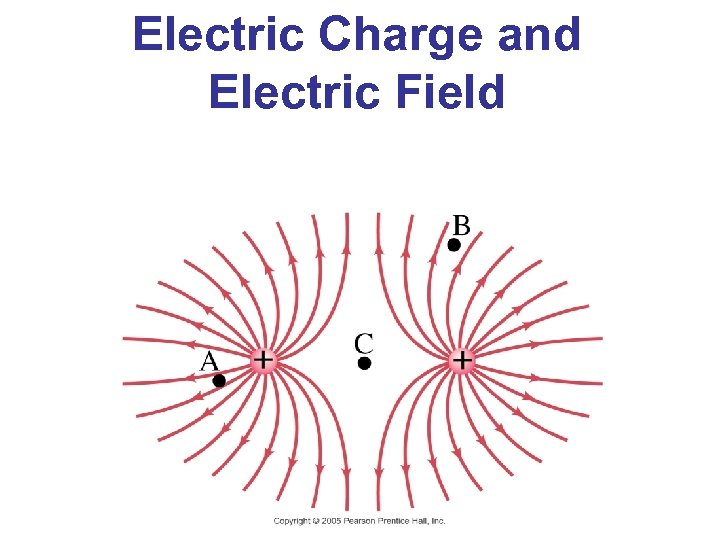Electric Charge and Electric Field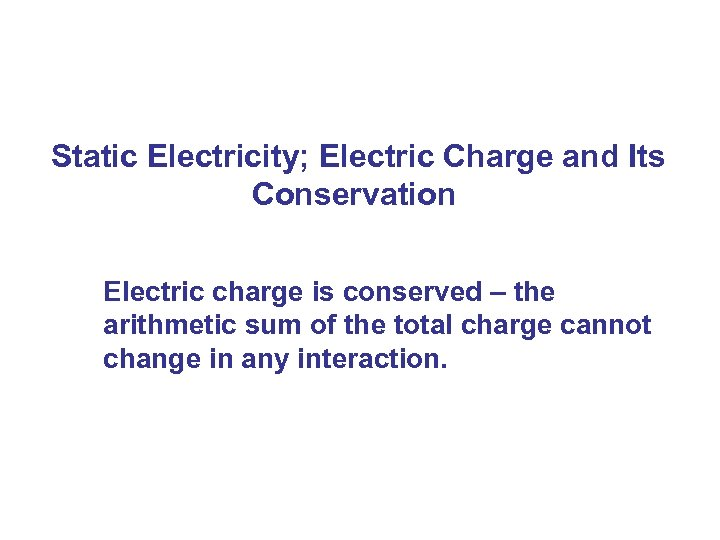Static Electricity; Electric Charge and Its Conservation Electric charge is conserved – the arithmetic sum of the total charge cannot change in any interaction.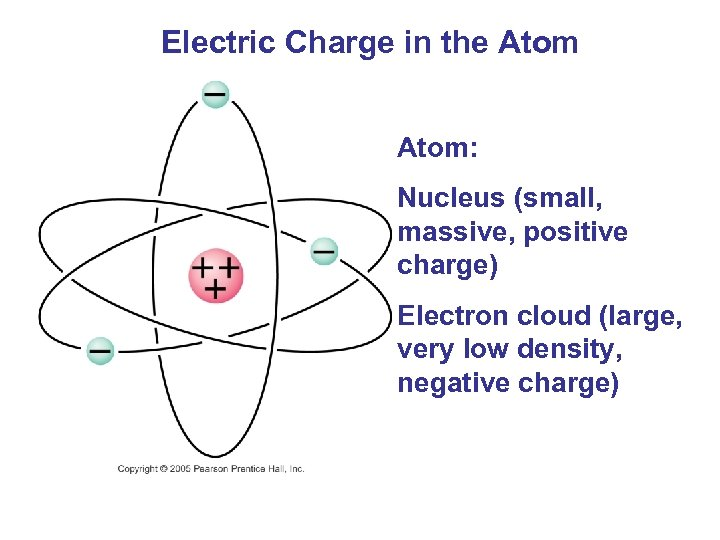Electric Charge in the Atom: Nucleus (small, massive, positive charge) Electron cloud (large, very low density, negative charge)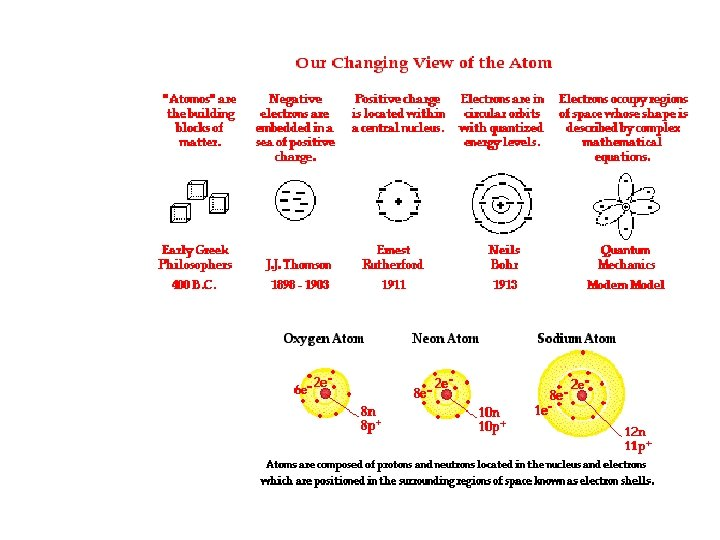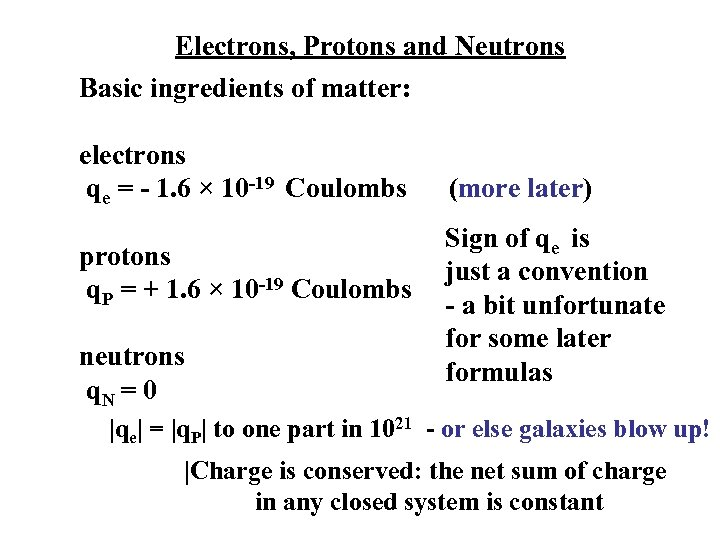Electrons, Protons and Neutrons Basic ingredients of matter: electrons qe = - 1. 6 × 10 -19 Coulombs protons q. P = + 1. 6 × 10 -19 Coulombs neutrons q. N = 0 (more later) Sign of qe is just a convention - a bit unfortunate for some later formulas |qe| = |q. P| to one part in 1021 - or else galaxies blow up! |Charge is conserved: the net sum of charge in any closed system is constant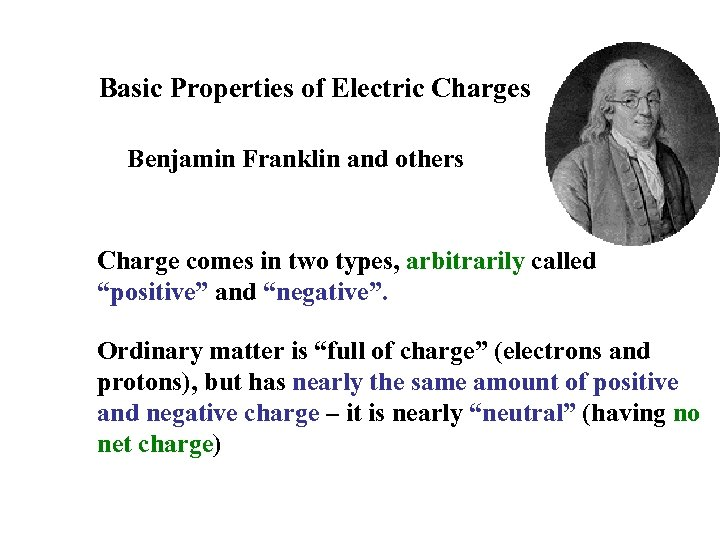Basic Properties of Electric Charges Benjamin Franklin and others Charge comes in two types, arbitrarily called “positive” and “negative”. Ordinary matter is “full of charge” (electrons and protons), but has nearly the same amount of positive and negative charge – it is nearly “neutral” (having no net charge)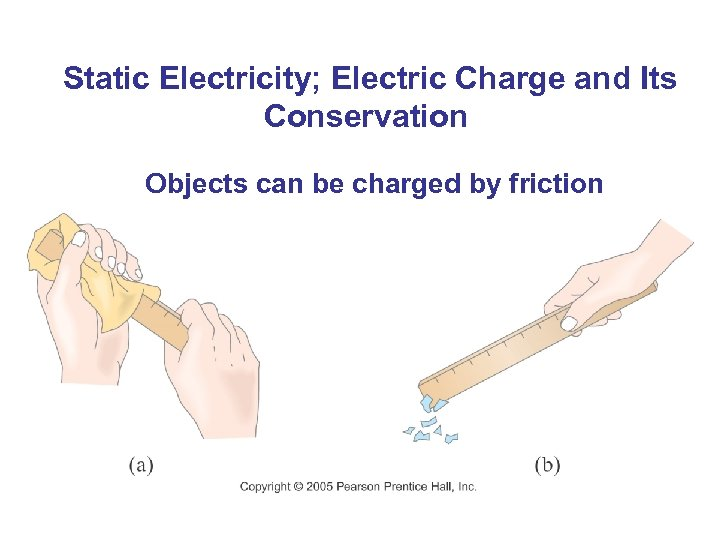Static Electricity; Electric Charge and Its Conservation Objects can be charged by friction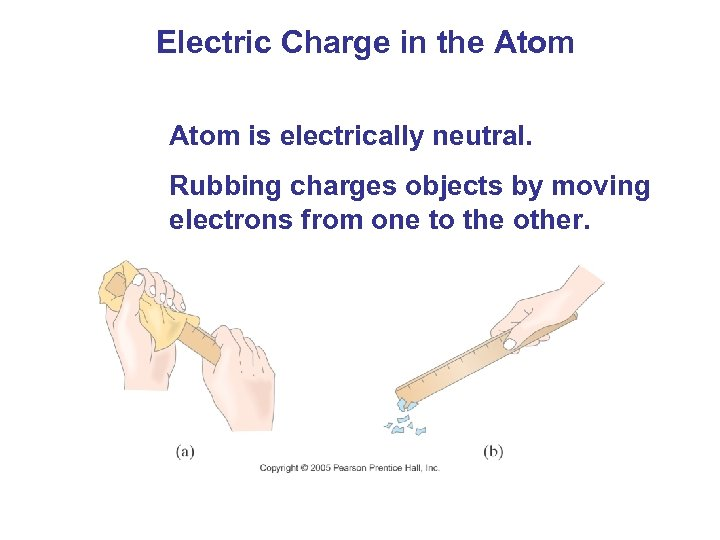Electric Charge in the Atom is electrically neutral. Rubbing charges objects by moving electrons from one to the other.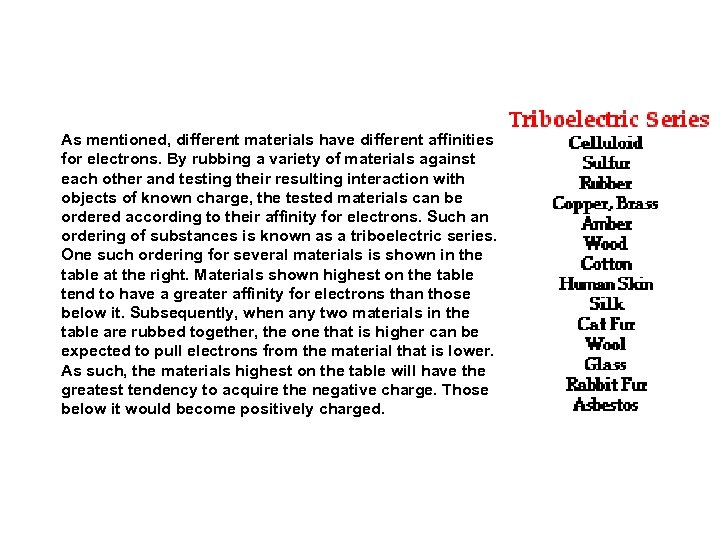As mentioned, different materials have different affinities for electrons. By rubbing a variety of materials against each other and testing their resulting interaction with objects of known charge, the tested materials can be ordered according to their affinity for electrons. Such an ordering of substances is known as a triboelectric series. One such ordering for several materials is shown in the table at the right. Materials shown highest on the table tend to have a greater affinity for electrons than those below it. Subsequently, when any two materials in the table are rubbed together, the one that is higher can be expected to pull electrons from the material that is lower. As such, the materials highest on the table will have the greatest tendency to acquire the negative charge. Those below it would become positively charged.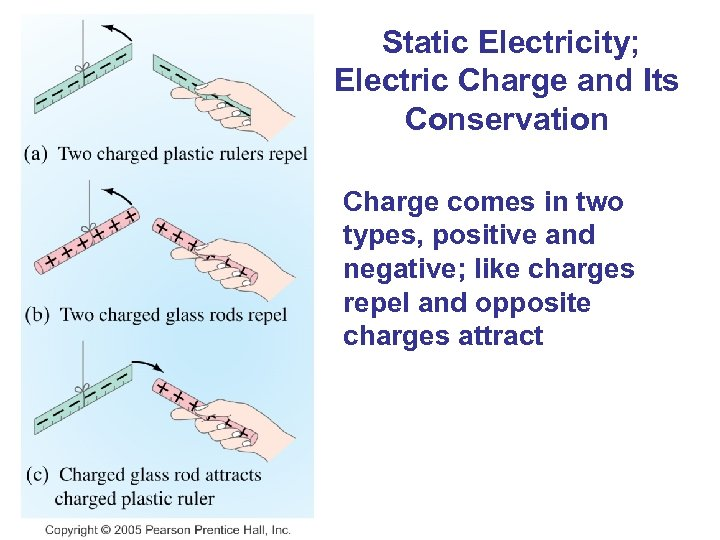Static Electricity; Electric Charge and Its Conservation Charge comes in two types, positive and negative; like charges repel and opposite charges attract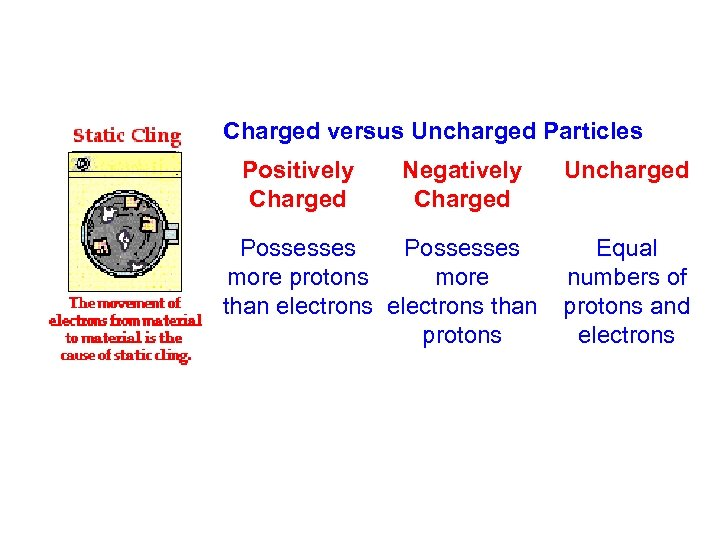Charged versus Uncharged Particles Positively Charged Negatively Charged Uncharged Possesses Equal more protons more numbers of than electrons than protons and protons electrons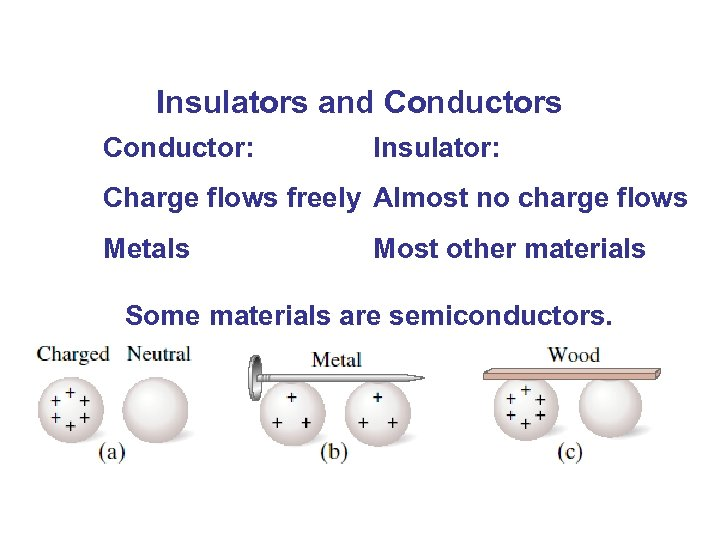Insulators and Conductors Conductor: Insulator: Charge flows freely Almost no charge flows Metals Most other materials Some materials are semiconductors.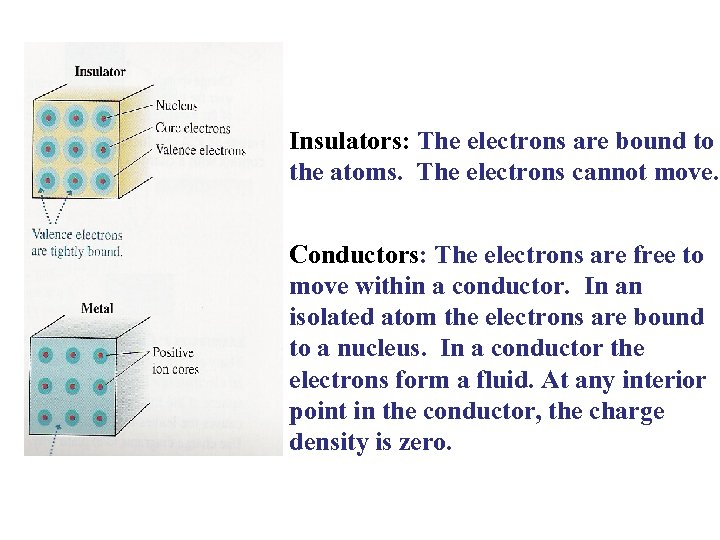Insulators: The electrons are bound to the atoms. The electrons cannot move. Conductors: The electrons are free to move within a conductor. In an isolated atom the electrons are bound to a nucleus. In a conductor the electrons form a fluid. At any interior point in the conductor, the charge density is zero.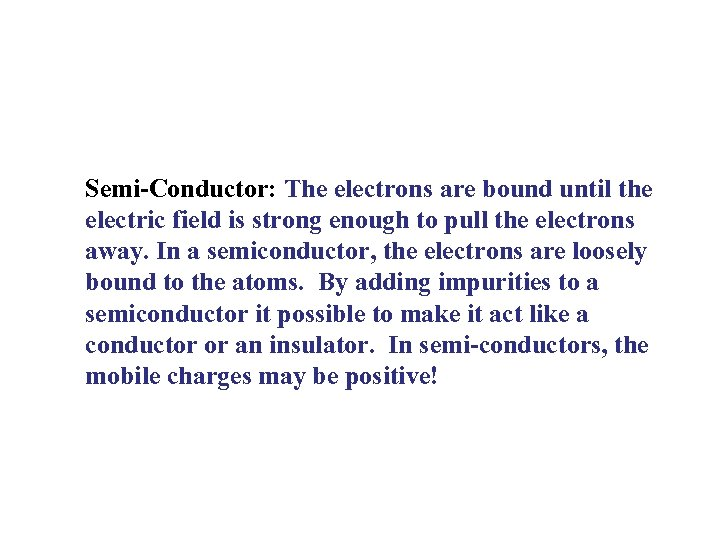Semi-Conductor: The electrons are bound until the electric field is strong enough to pull the electrons away. In a semiconductor, the electrons are loosely bound to the atoms. By adding impurities to a semiconductor it possible to make it act like a conductor or an insulator. In semi-conductors, the mobile charges may be positive!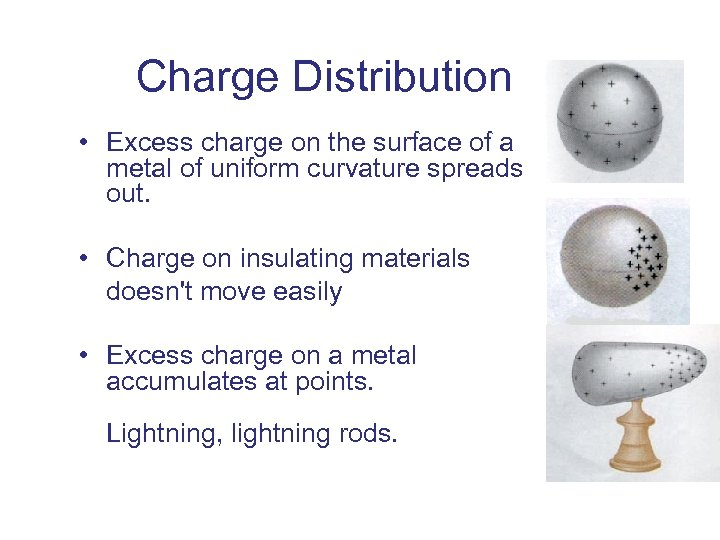Charge Distribution • Excess charge on the surface of a metal of uniform curvature spreads out. • Charge on insulating materials doesn't move easily • Excess charge on a metal accumulates at points. Lightning, lightning rods.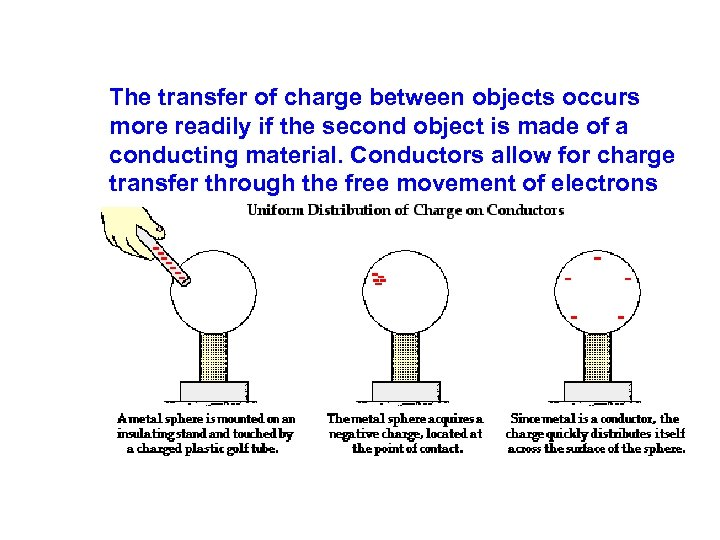The transfer of charge between objects occurs more readily if the second object is made of a conducting material. Conductors allow for charge transfer through the free movement of electrons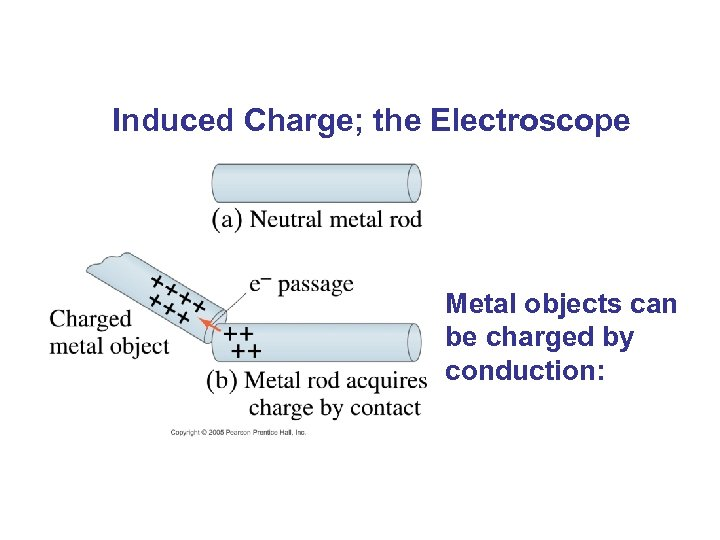Induced Charge; the Electroscope Metal objects can be charged by conduction: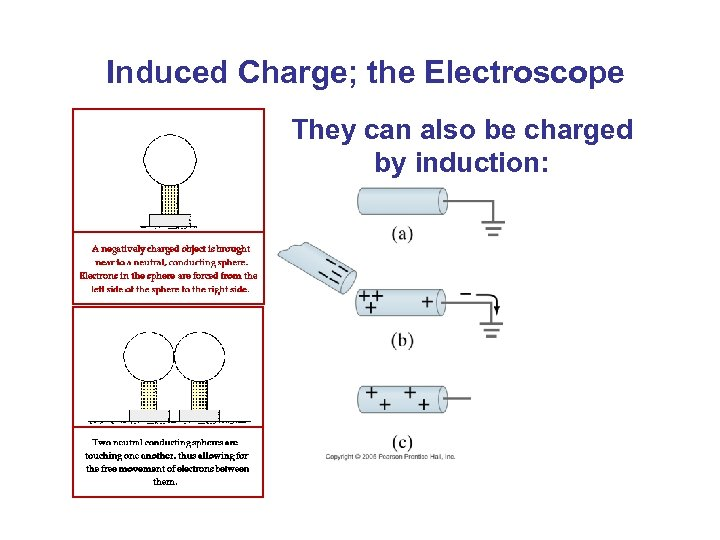Induced Charge; the Electroscope They can also be charged by induction: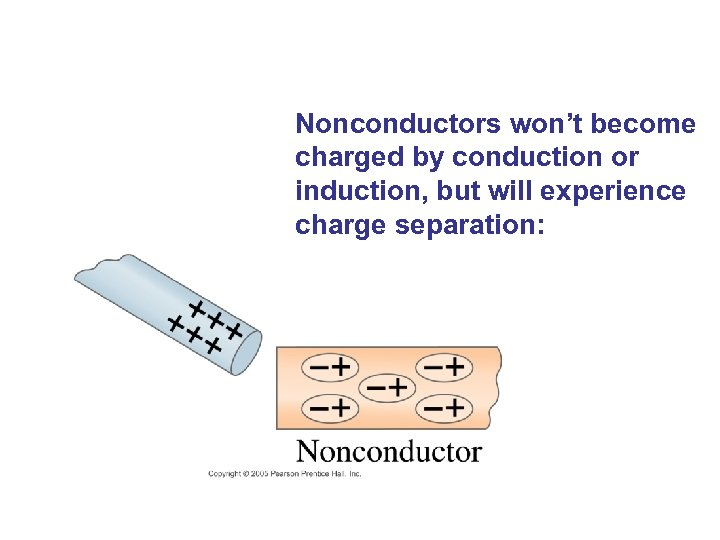Nonconductors won’t become charged by conduction or induction, but will experience charge separation: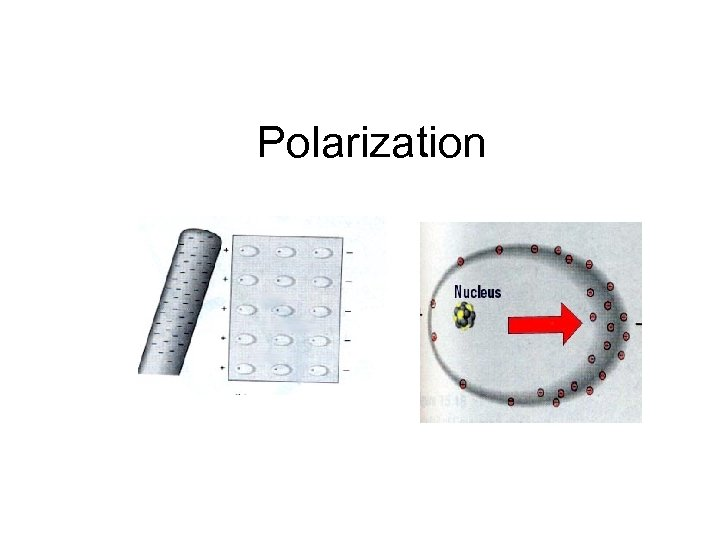Polarization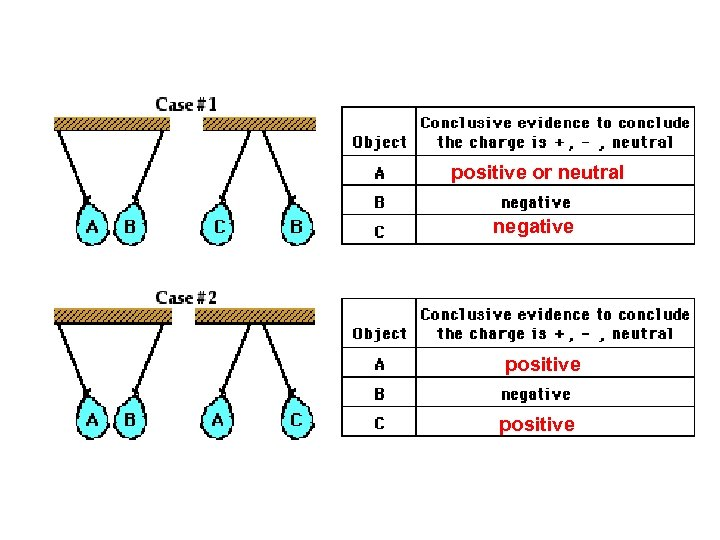positive or neutral negative positive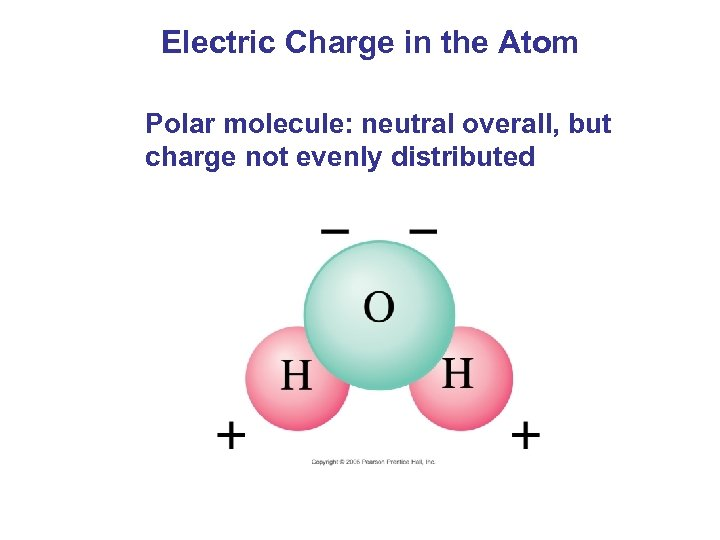Electric Charge in the Atom Polar molecule: neutral overall, but charge not evenly distributed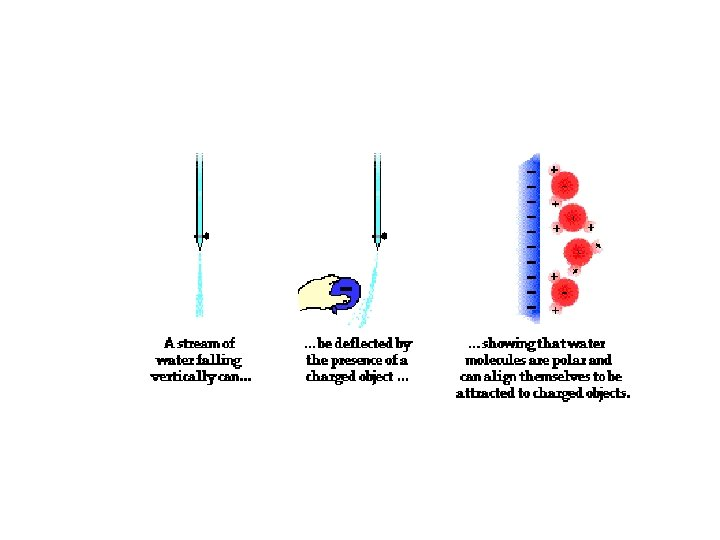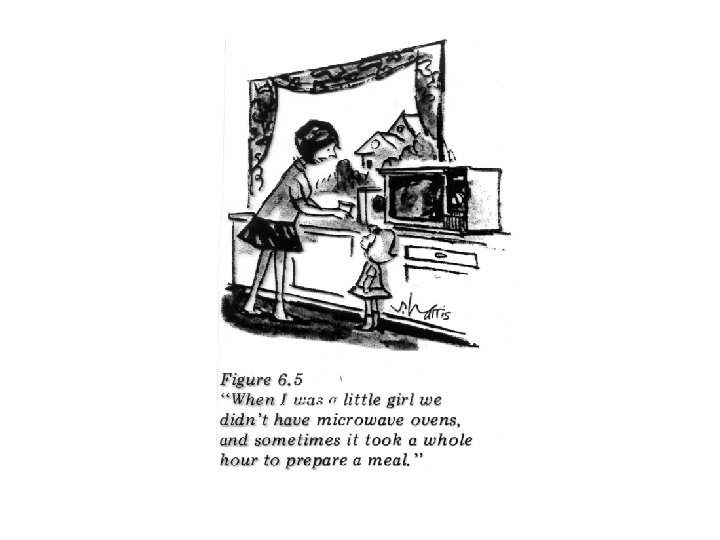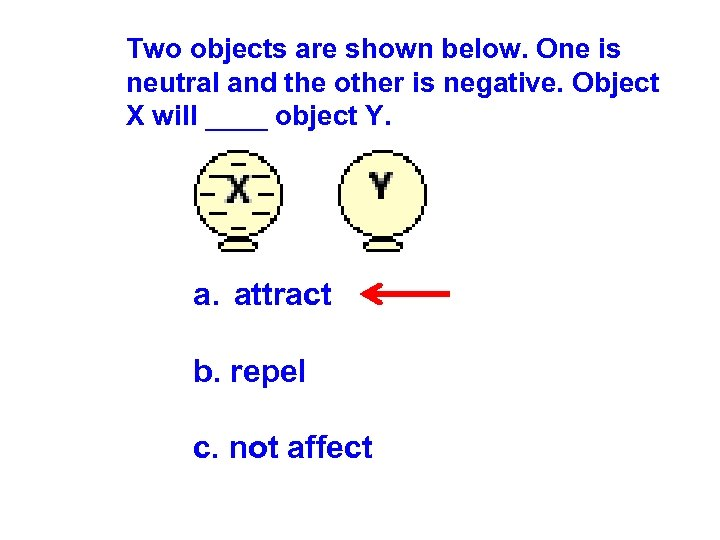Two objects are shown below. One is neutral and the other is negative. Object X will ____ object Y. a. attract b. repel c. not affect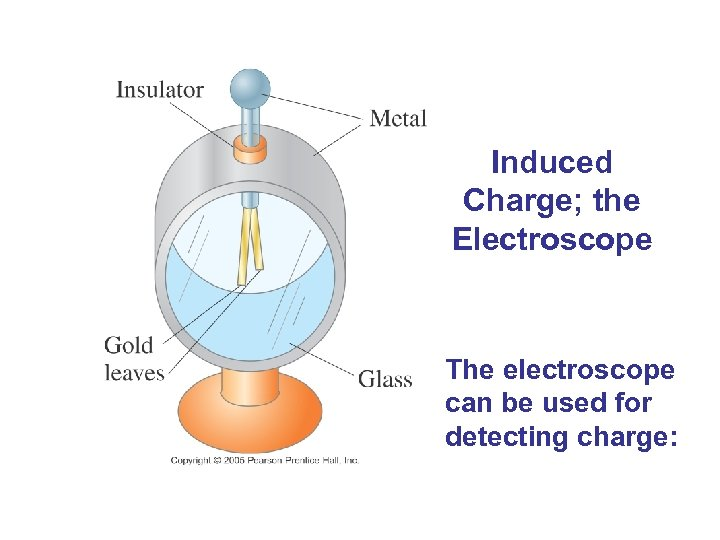Induced Charge; the Electroscope The electroscope can be used for detecting charge: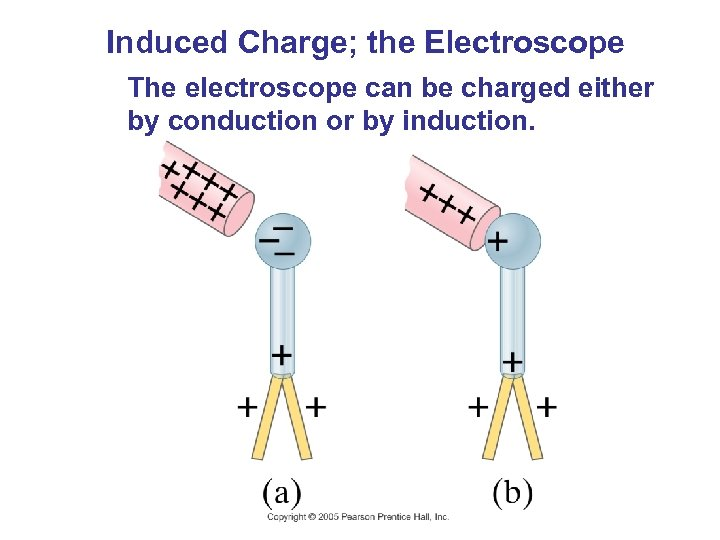Induced Charge; the Electroscope The electroscope can be charged either by conduction or by induction.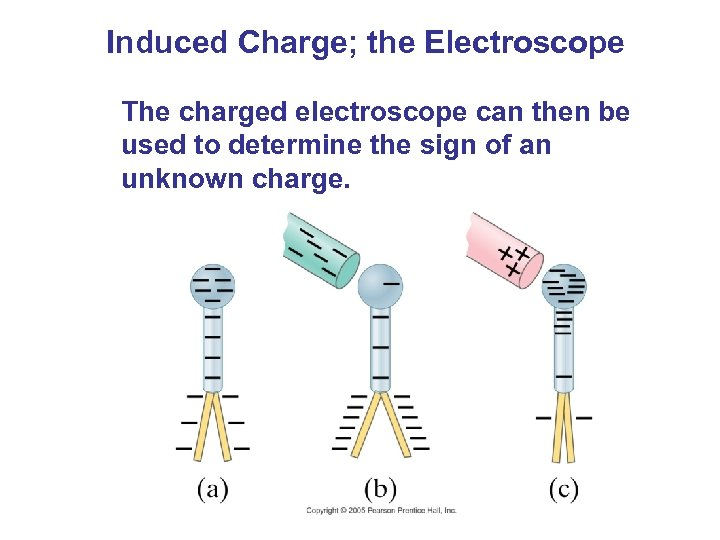Induced Charge; the Electroscope The charged electroscope can then be used to determine the sign of an unknown charge.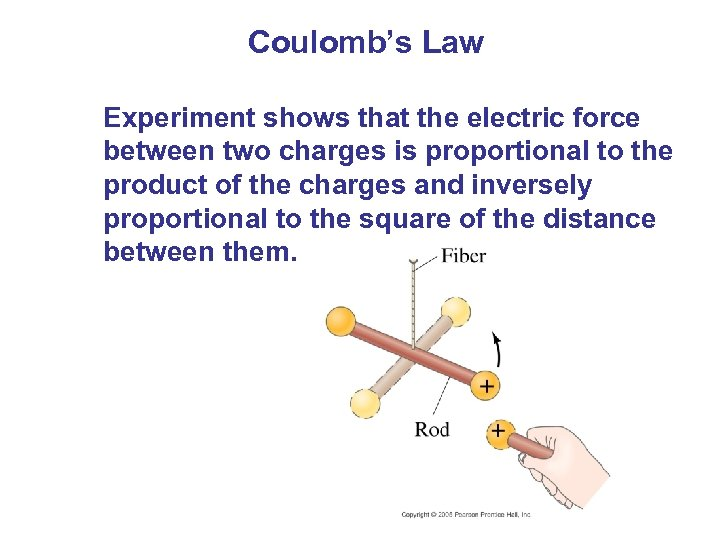Coulomb’s Law Experiment shows that the electric force between two charges is proportional to the product of the charges and inversely proportional to the square of the distance between them.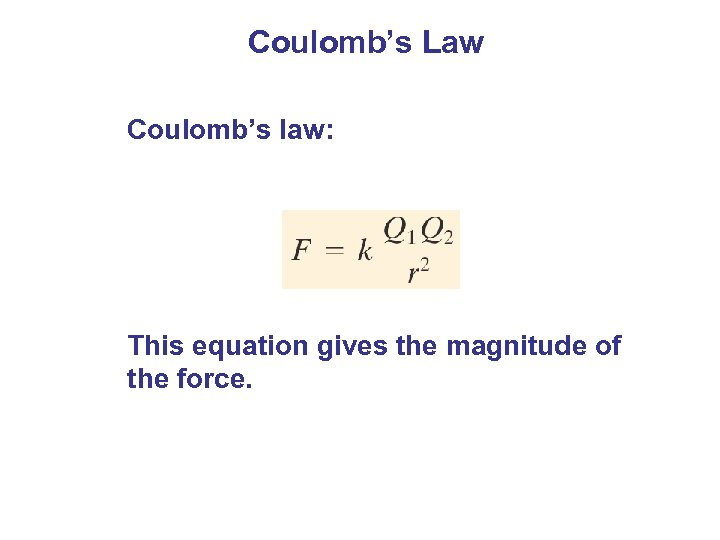Coulomb’s Law Coulomb’s law: This equation gives the magnitude of the force.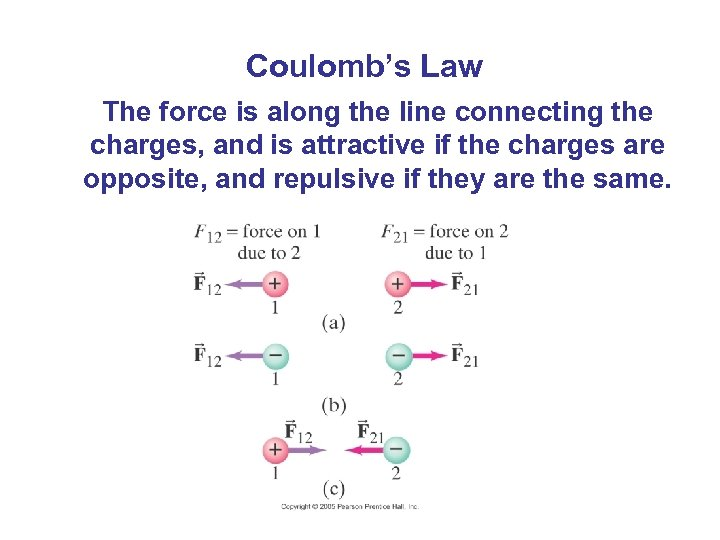Coulomb’s Law The force is along the line connecting the charges, and is attractive if the charges are opposite, and repulsive if they are the same.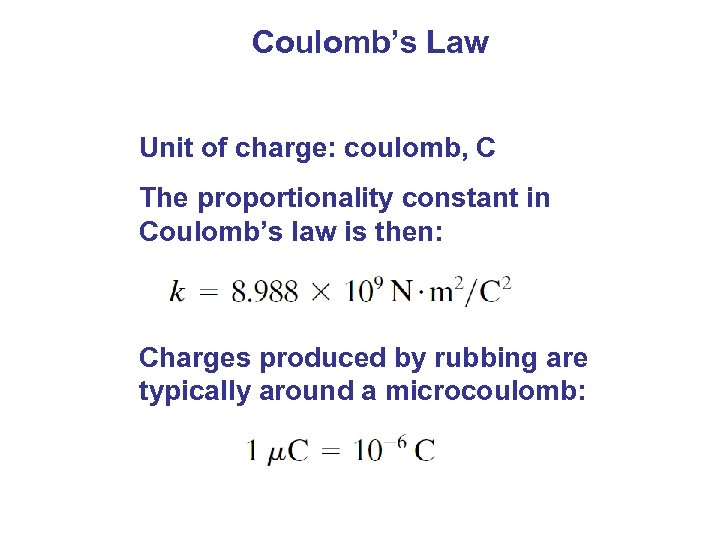Coulomb’s Law Unit of charge: coulomb, C The proportionality constant in Coulomb’s law is then: Charges produced by rubbing are typically around a microcoulomb: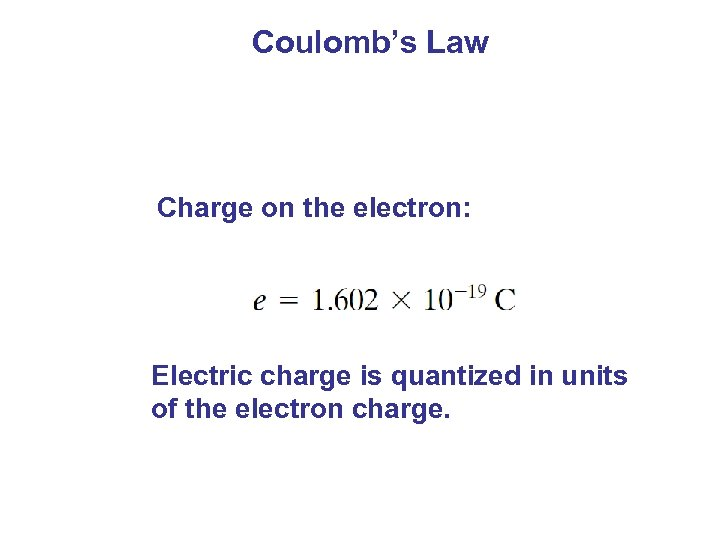Coulomb’s Law Charge on the electron: Electric charge is quantized in units of the electron charge.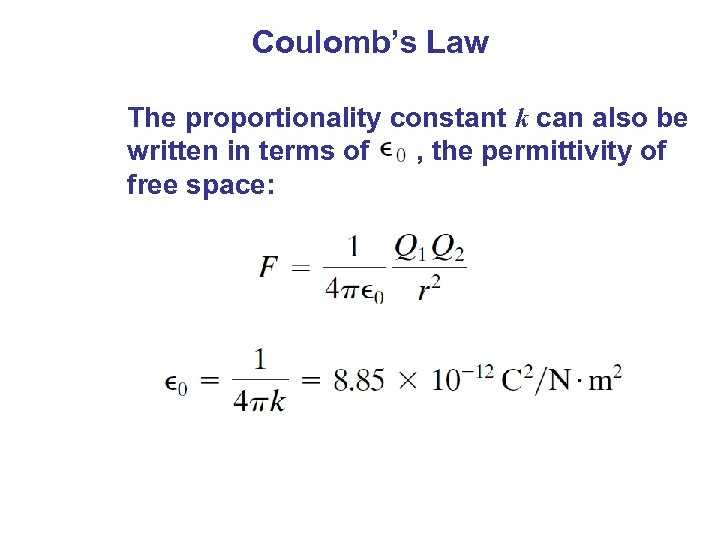Coulomb’s Law The proportionality constant k can also be written in terms of , the permittivity of free space: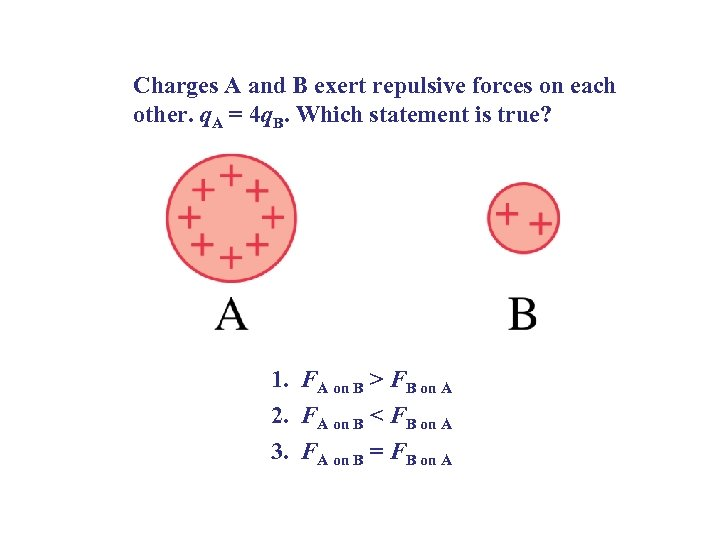Charges A and B exert repulsive forces on each other. q. A = 4 q. B. Which statement is true? 1. FA on B > FB on A 2. FA on B < FB on A 3. FA on B = FB on A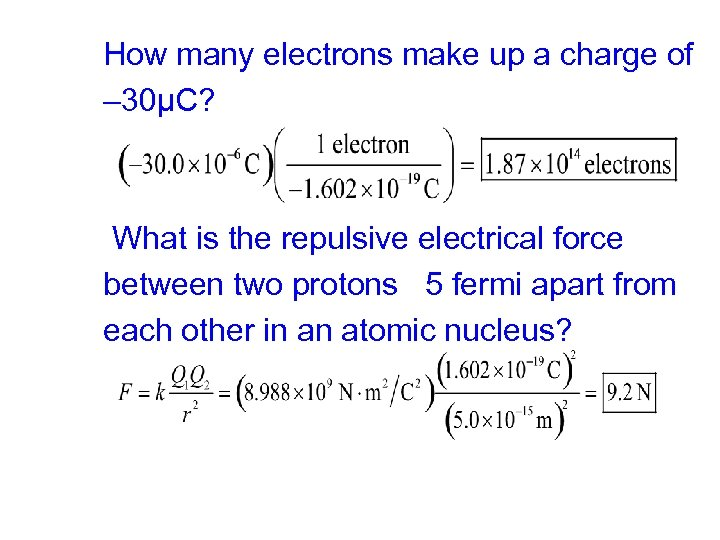How many electrons make up a charge of – 30μC? What is the repulsive electrical force between two protons 5 fermi apart from each other in an atomic nucleus?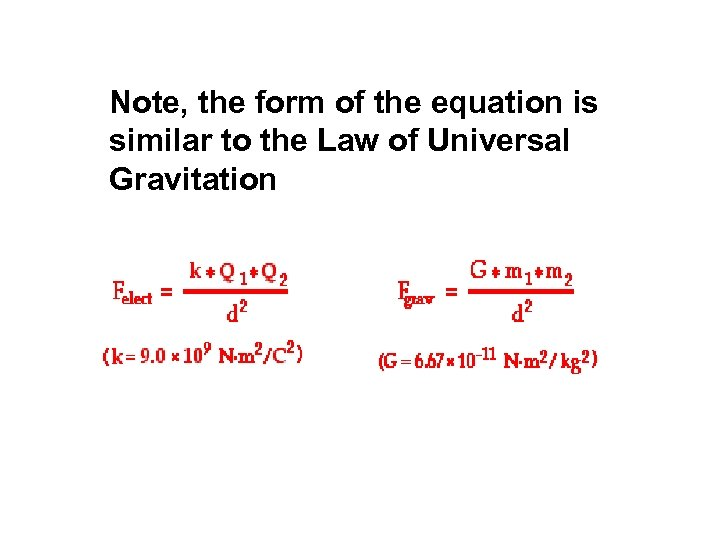Note, the form of the equation is similar to the Law of Universal Gravitation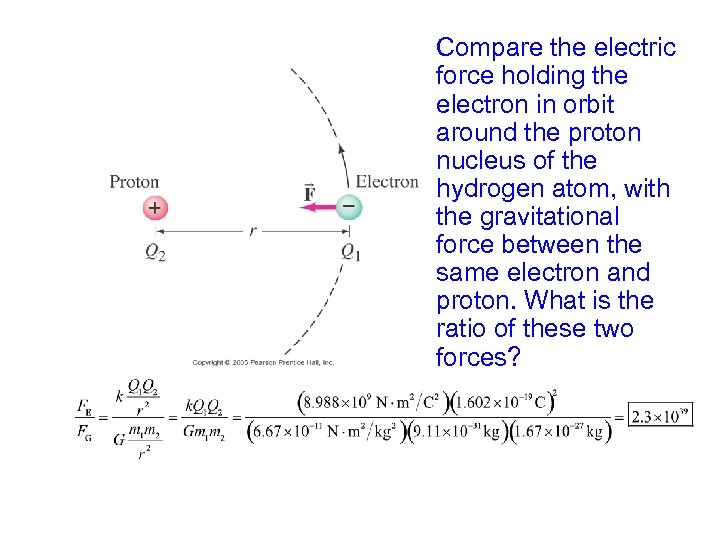Compare the electric force holding the electron in orbit around the proton nucleus of the hydrogen atom, with the gravitational force between the same electron and proton. What is the ratio of these two forces?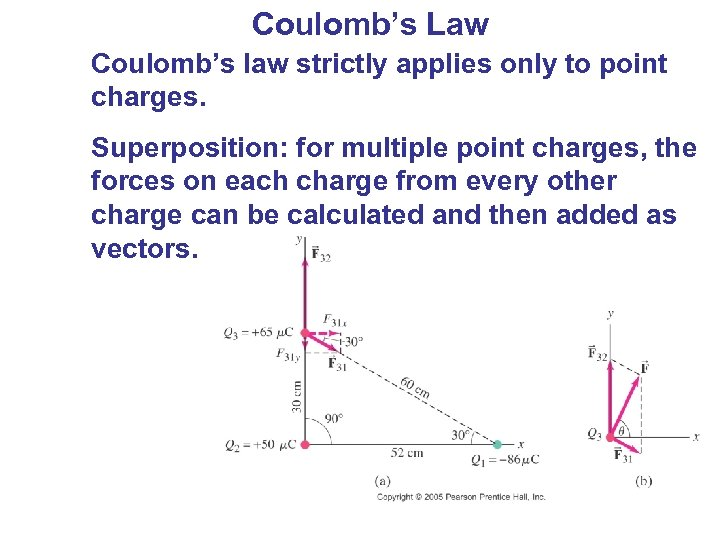Coulomb’s Law Coulomb’s law strictly applies only to point charges. Superposition: for multiple point charges, the forces on each charge from every other charge can be calculated and then added as vectors.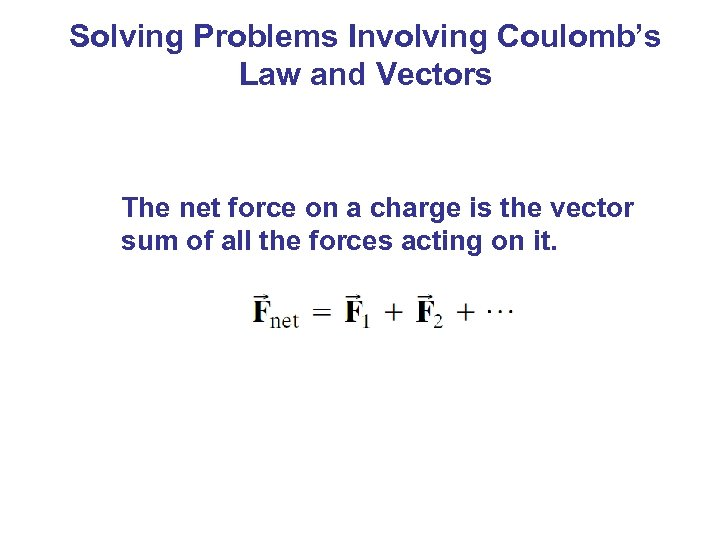Solving Problems Involving Coulomb’s Law and Vectors The net force on a charge is the vector sum of all the forces acting on it.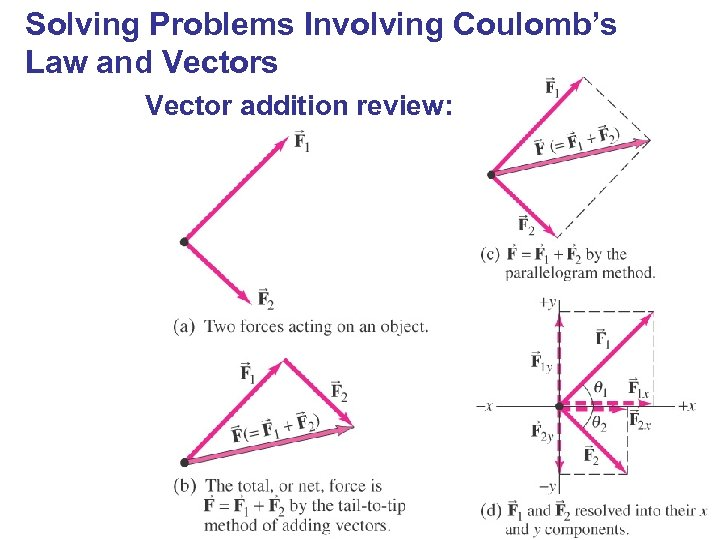Solving Problems Involving Coulomb’s Law and Vectors Vector addition review: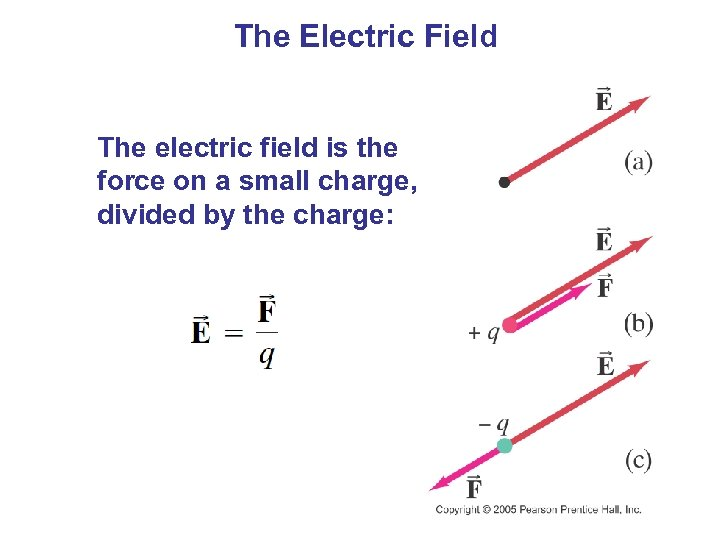The Electric Field The electric field is the force on a small charge, divided by the charge: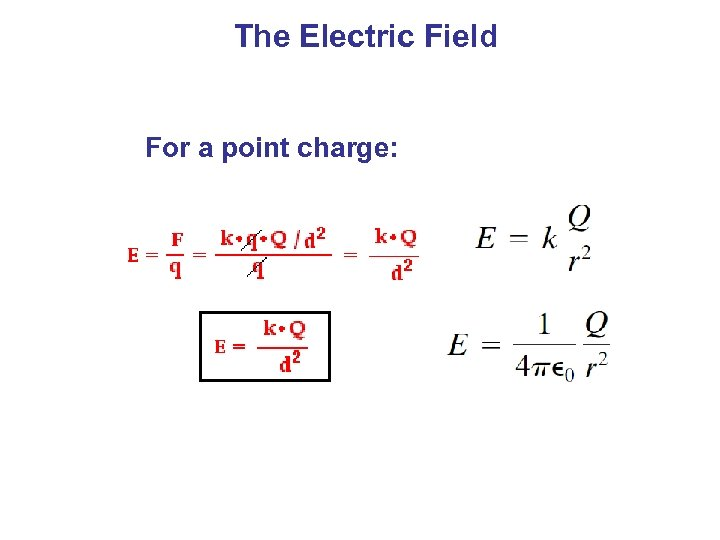The Electric Field For a point charge: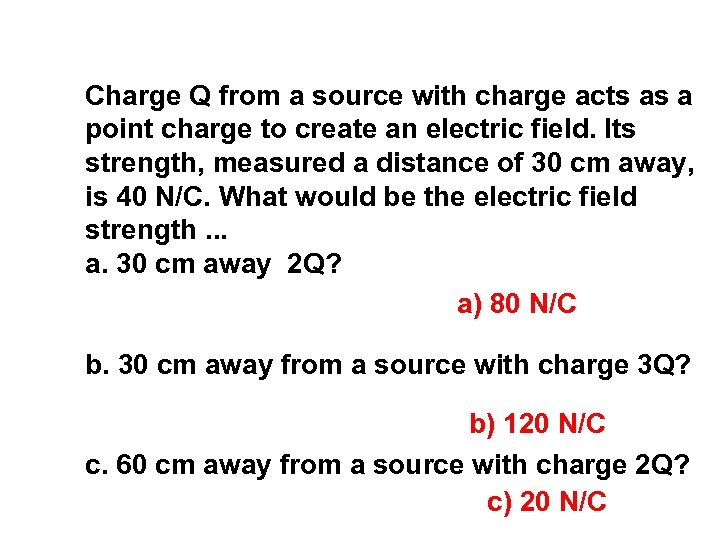Charge Q from a source with charge acts as a point charge to create an electric field. Its strength, measured a distance of 30 cm away, is 40 N/C. What would be the electric field strength. . . a. 30 cm away 2 Q? a) 80 N/C b. 30 cm away from a source with charge 3 Q? b) 120 N/C c. 60 cm away from a source with charge 2 Q? c) 20 N/C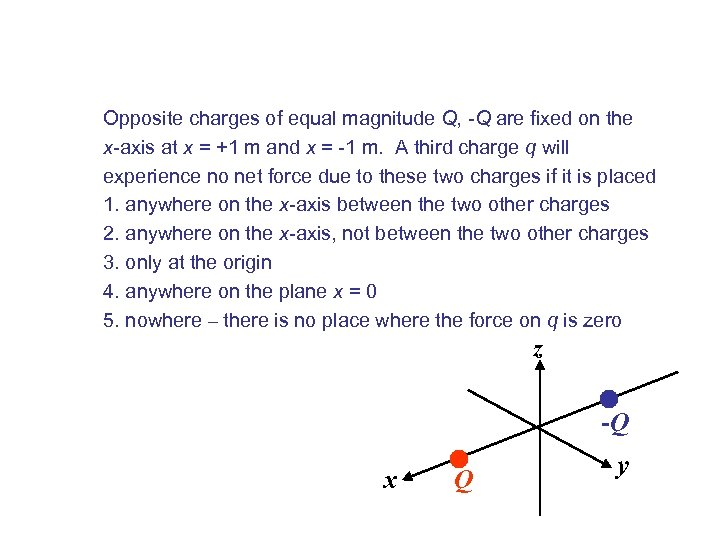Opposite charges of equal magnitude Q, -Q are fixed on the x-axis at x = +1 m and x = -1 m. A third charge q will experience no net force due to these two charges if it is placed 1. anywhere on the x-axis between the two other charges 2. anywhere on the x-axis, not between the two other charges 3. only at the origin 4. anywhere on the plane x = 0 5. nowhere – there is no place where the force on q is zero z -Q x Q y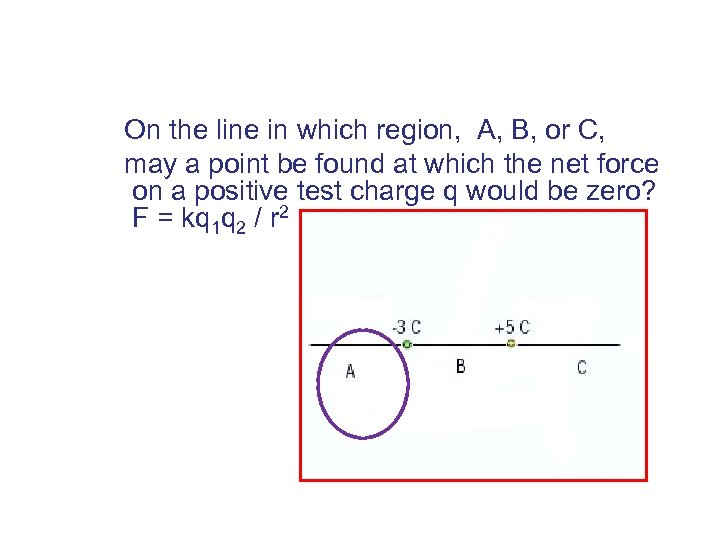On the line in which region, A, B, or C, may a point be found at which the net force on a positive test charge q would be zero? F = kq 1 q 2 / r 2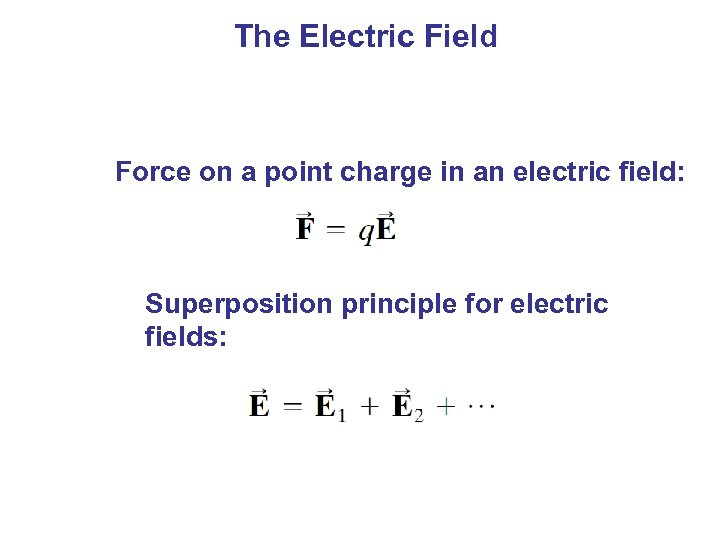The Electric Field Force on a point charge in an electric field: Superposition principle for electric fields: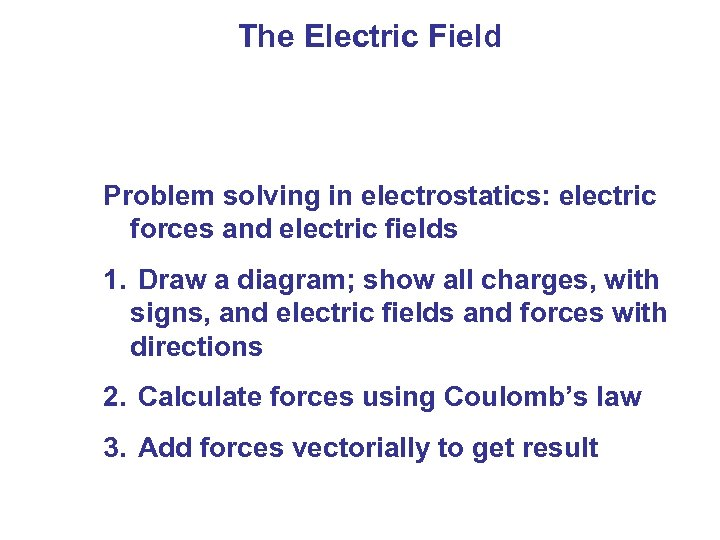The Electric Field Problem solving in electrostatics: electric forces and electric fields 1. Draw a diagram; show all charges, with signs, and electric fields and forces with directions 2. Calculate forces using Coulomb’s law 3. Add forces vectorially to get result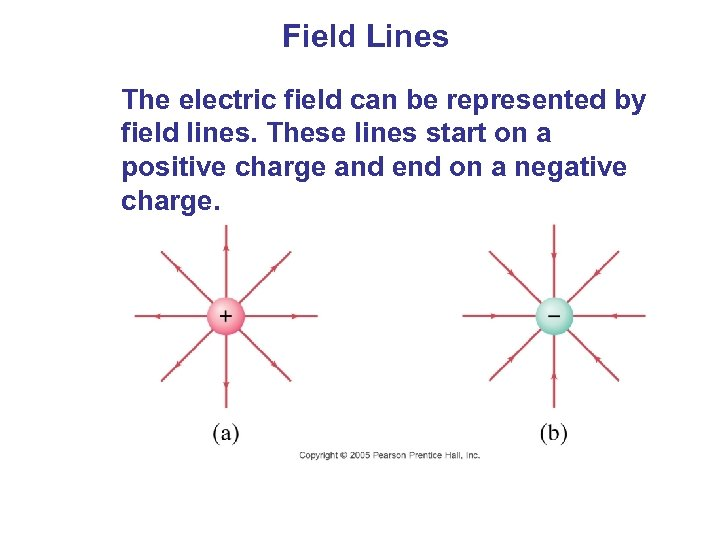Field Lines The electric field can be represented by field lines. These lines start on a positive charge and end on a negative charge.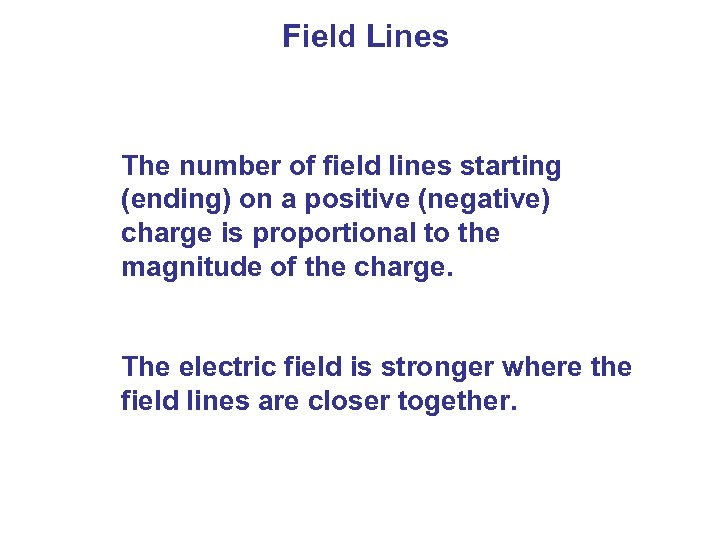Field Lines The number of field lines starting (ending) on a positive (negative) charge is proportional to the magnitude of the charge. The electric field is stronger where the field lines are closer together.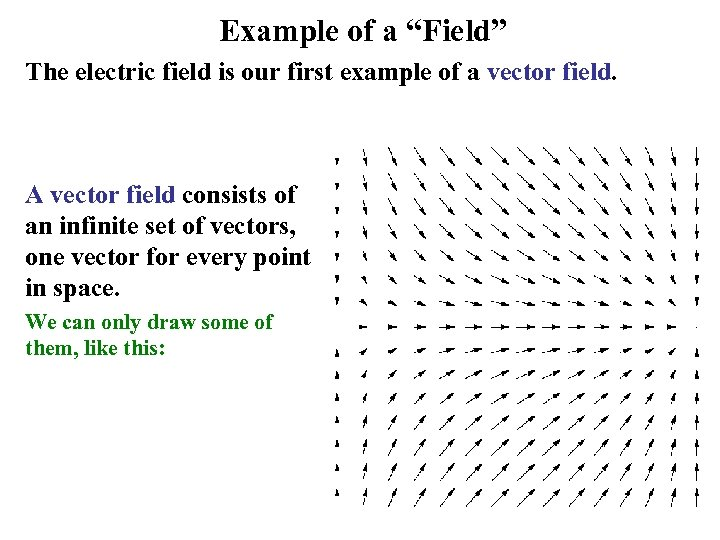Example of a “Field” The electric field is our first example of a vector field. A vector field consists of an infinite set of vectors, one vector for every point in space. We can only draw some of them, like this: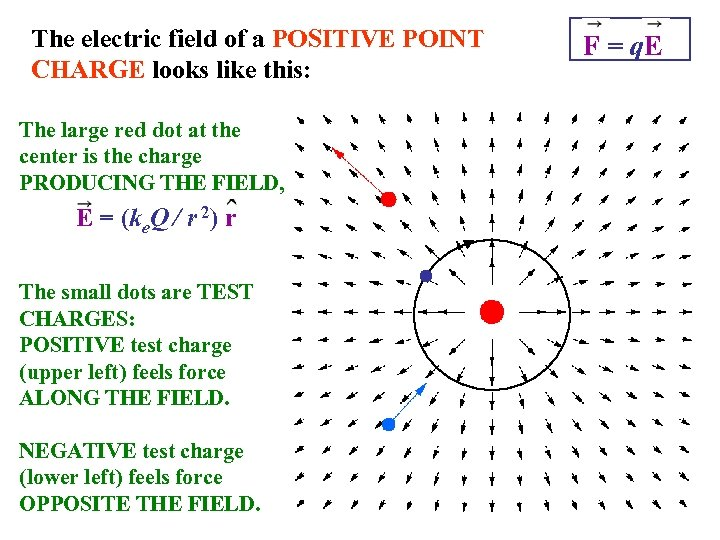The electric field of a POSITIVE POINT CHARGE looks like this: The large red dot at the center is the charge PRODUCING THE FIELD, E = (ke. Q / r 2) r The small dots are TEST CHARGES: POSITIVE test charge (upper left) feels force ALONG THE FIELD. NEGATIVE test charge (lower left) feels force OPPOSITE THE FIELD. F = q. E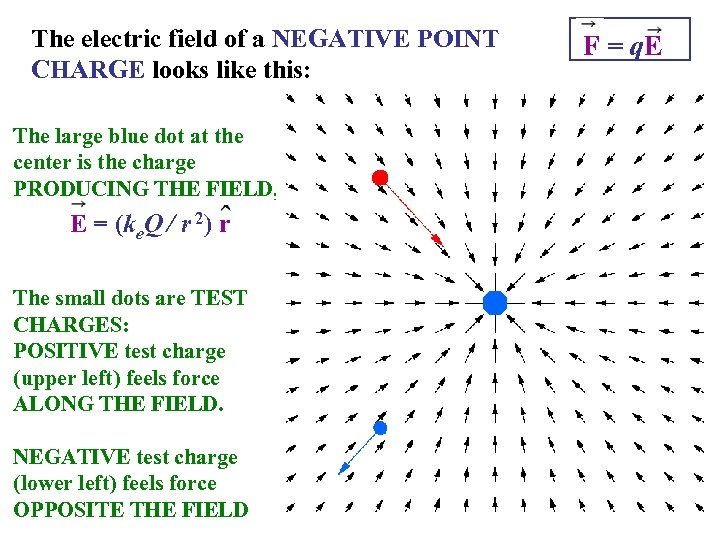The electric field of a NEGATIVE POINT CHARGE looks like this: The large blue dot at the center is the charge PRODUCING THE FIELD, E = (ke. Q / r 2) r The small dots are TEST CHARGES: POSITIVE test charge (upper left) feels force ALONG THE FIELD. NEGATIVE test charge (lower left) feels force OPPOSITE THE FIELD F = q. E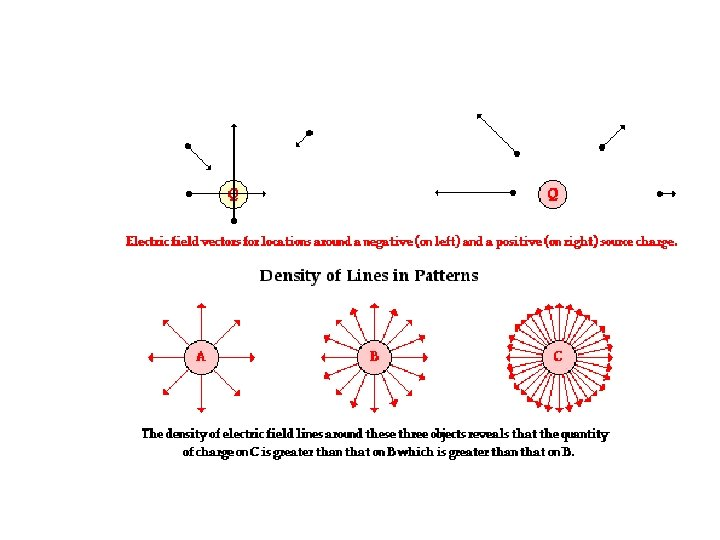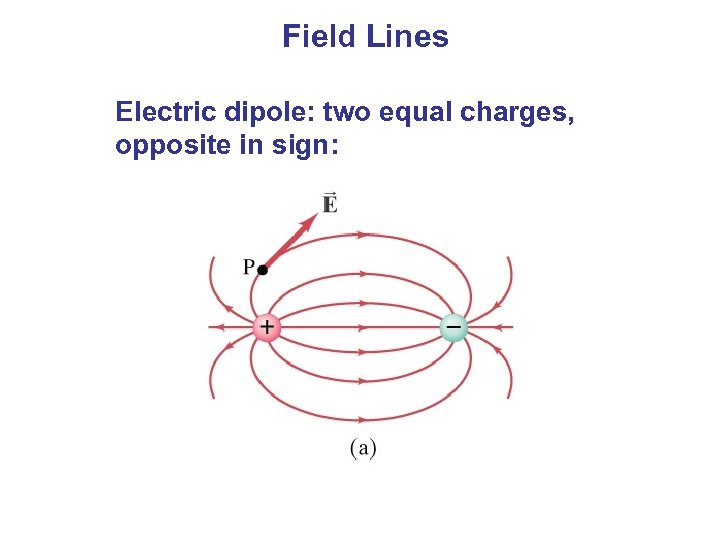Field Lines Electric dipole: two equal charges, opposite in sign: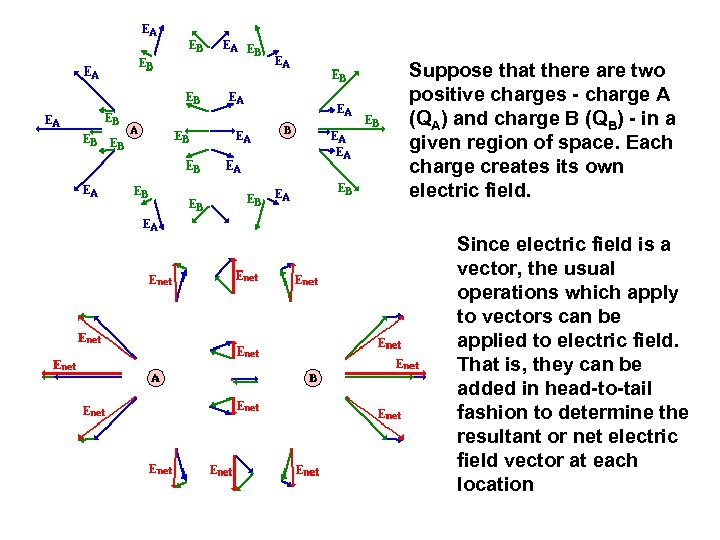Suppose that there are two positive charges - charge A (QA) and charge B (QB) - in a given region of space. Each charge creates its own electric field. Since electric field is a vector, the usual operations which apply to vectors can be applied to electric field. That is, they can be added in head-to-tail fashion to determine the resultant or net electric field vector at each location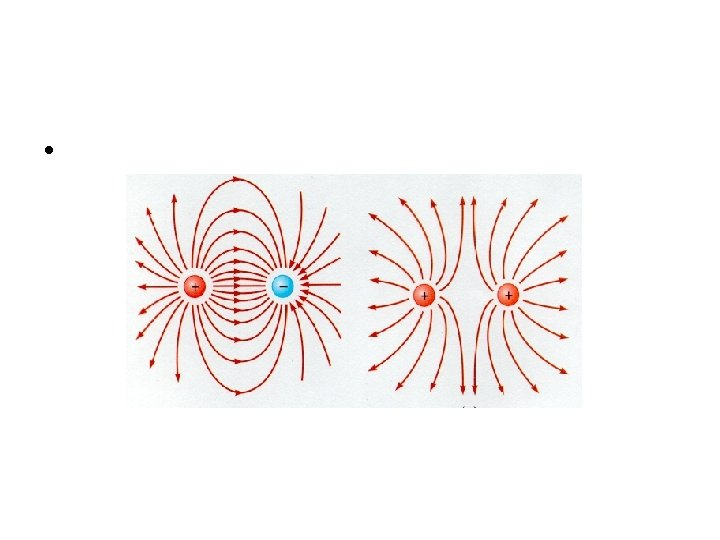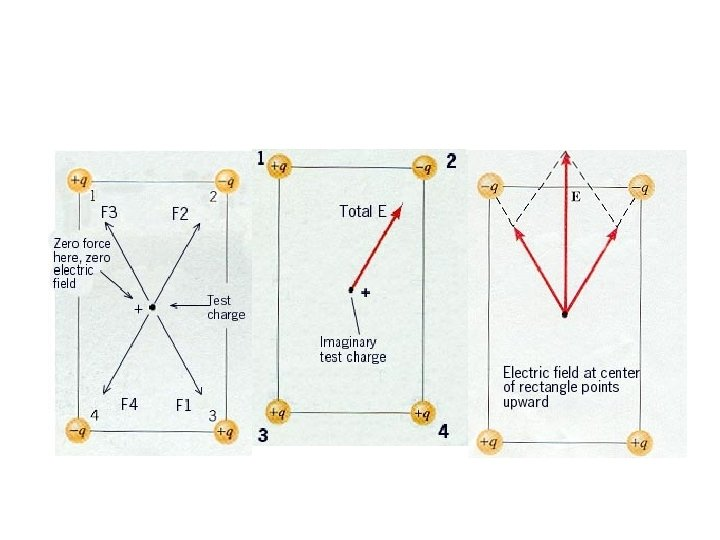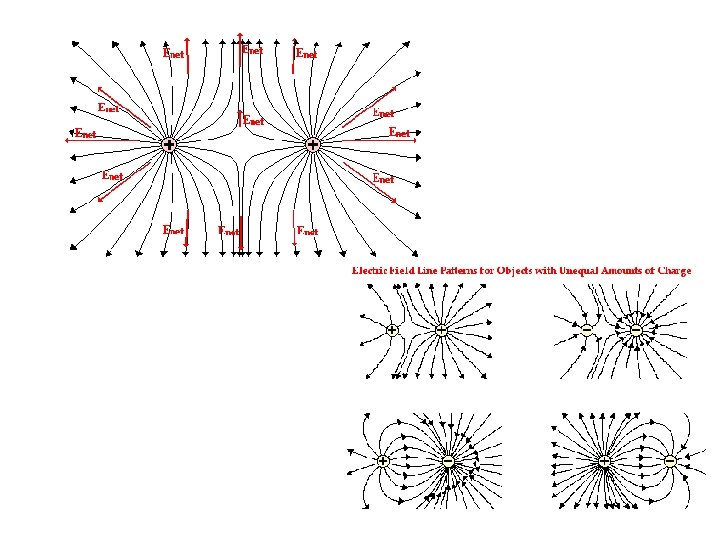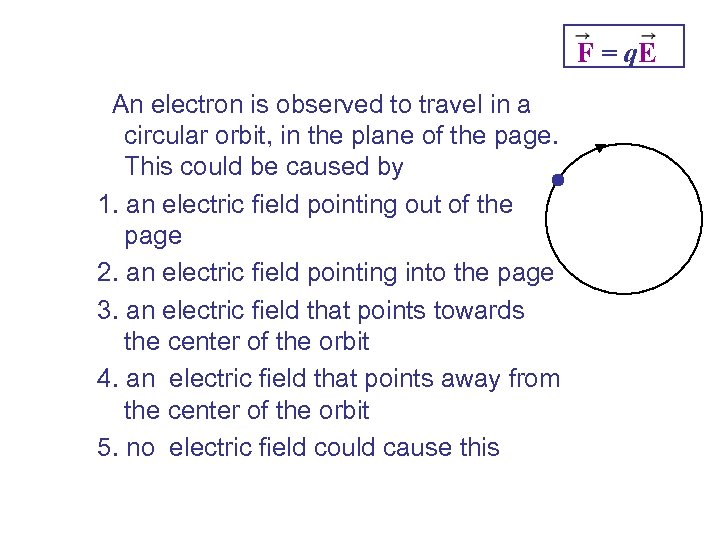F = q. E An electron is observed to travel in a circular orbit, in the plane of the page. This could be caused by 1. an electric field pointing out of the page 2. an electric field pointing into the page 3. an electric field that points towards the center of the orbit 4. an electric field that points away from the center of the orbit 5. no electric field could cause this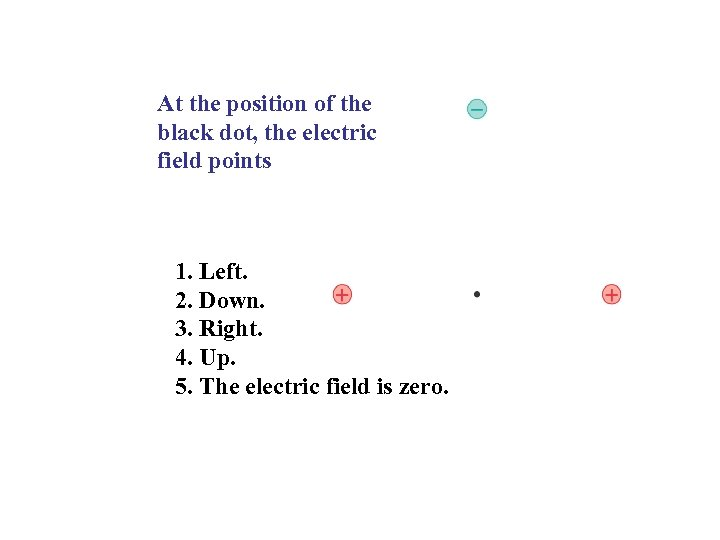At the position of the black dot, the electric field points 1. Left. 2. Down. 3. Right. 4. Up. 5. The electric field is zero.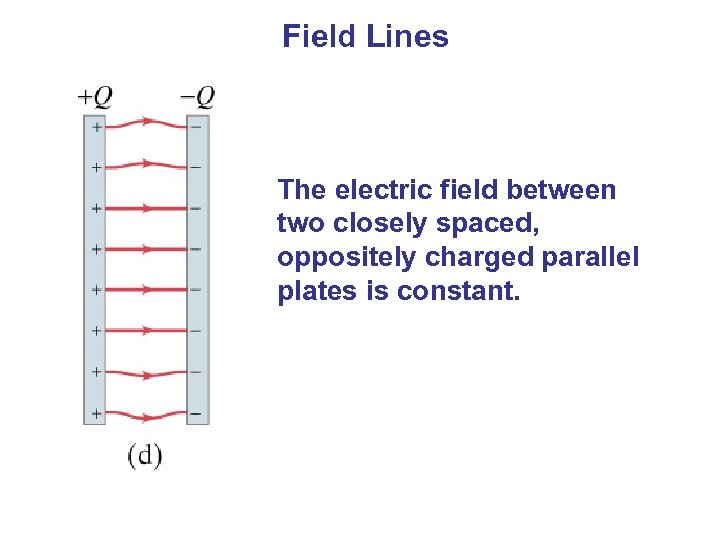Field Lines The electric field between two closely spaced, oppositely charged parallel plates is constant.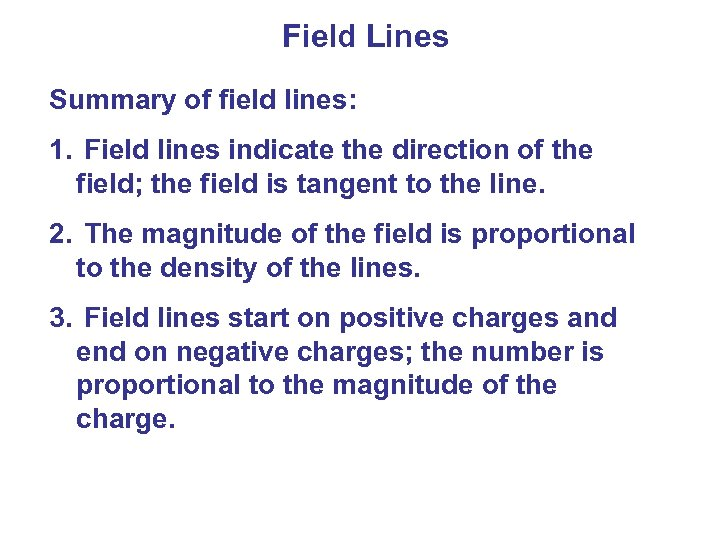Field Lines Summary of field lines: 1. Field lines indicate the direction of the field; the field is tangent to the line. 2. The magnitude of the field is proportional to the density of the lines. 3. Field lines start on positive charges and end on negative charges; the number is proportional to the magnitude of the charge.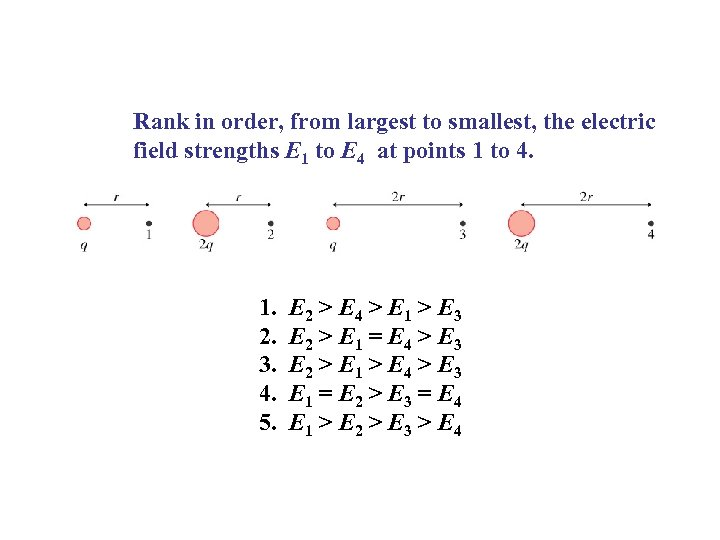Rank in order, from largest to smallest, the electric field strengths E 1 to E 4 at points 1 to 4. 1. 2. 3. 4. 5. E 2 > E 4 > E 1 > E 3 E 2 > E 1 = E 4 > E 3 E 2 > E 1 > E 4 > E 3 E 1 = E 2 > E 3 = E 4 E 1 > E 2 > E 3 > E 4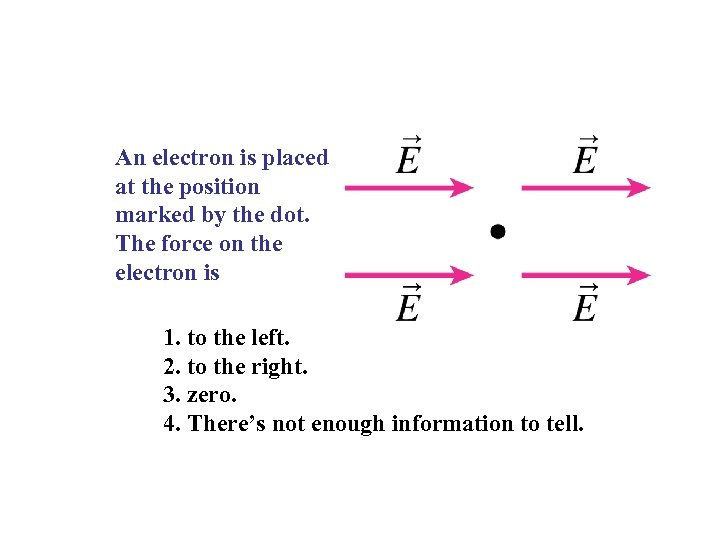An electron is placed at the position marked by the dot. The force on the electron is 1. to the left. 2. to the right. 3. zero. 4. There’s not enough information to tell.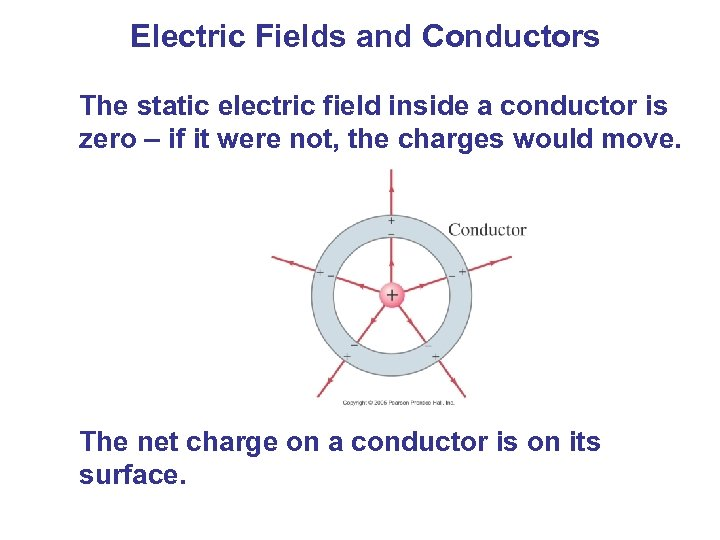Electric Fields and Conductors The static electric field inside a conductor is zero – if it were not, the charges would move. The net charge on a conductor is on its surface.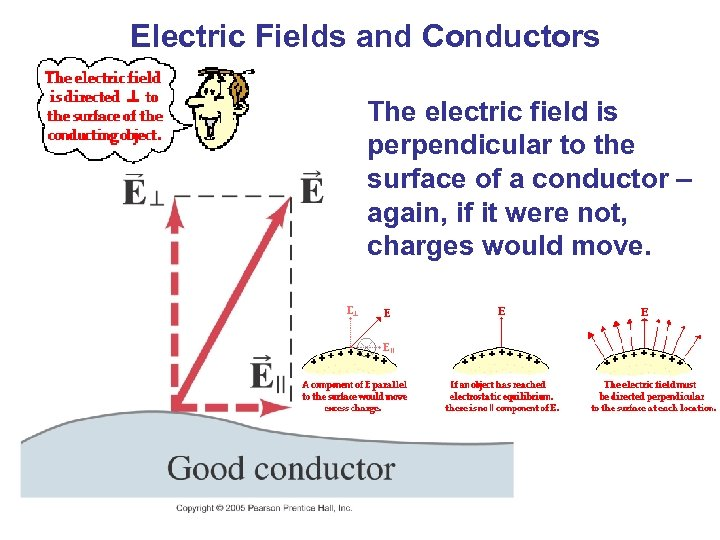Electric Fields and Conductors The electric field is perpendicular to the surface of a conductor – again, if it were not, charges would move.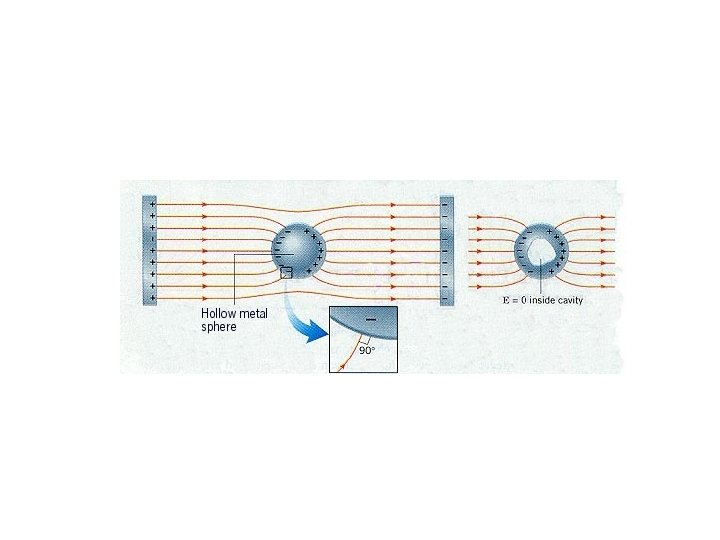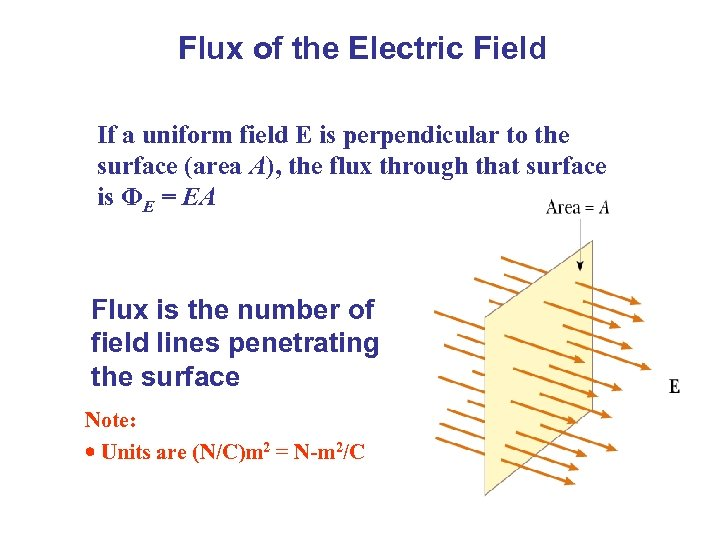Flux of the Electric Field If a uniform field E is perpendicular to the surface (area A), the flux through that surface is ΦE = EA Flux is the number of field lines penetrating the surface Note: Units are (N/C)m 2 = N-m 2/C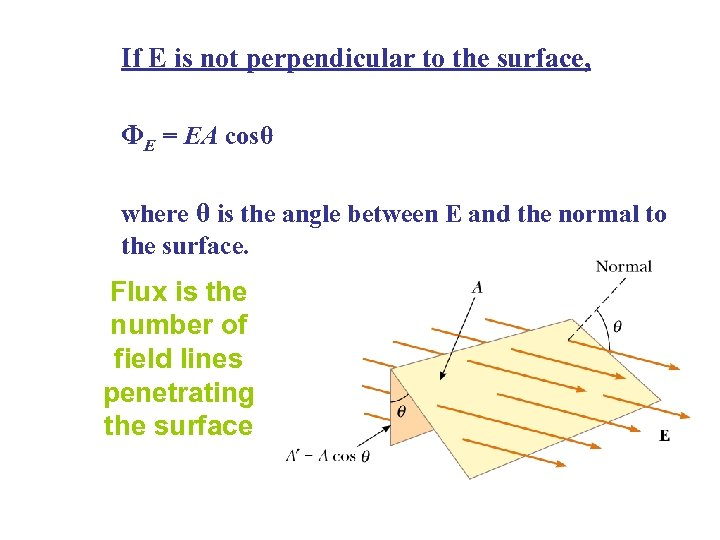If E is not perpendicular to the surface, ΦE = EA cosθ where θ is the angle between E and the normal to the surface. Flux is the number of field lines penetrating the surface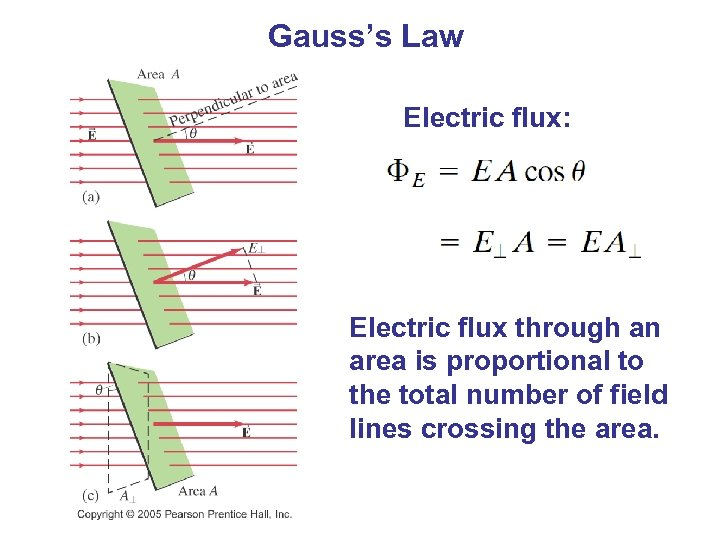Gauss’s Law Electric flux: Electric flux through an area is proportional to the total number of field lines crossing the area.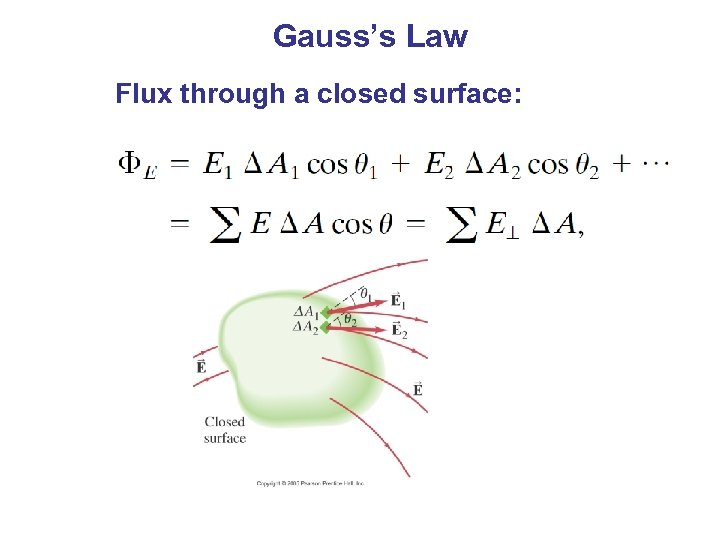Gauss’s Law Flux through a closed surface: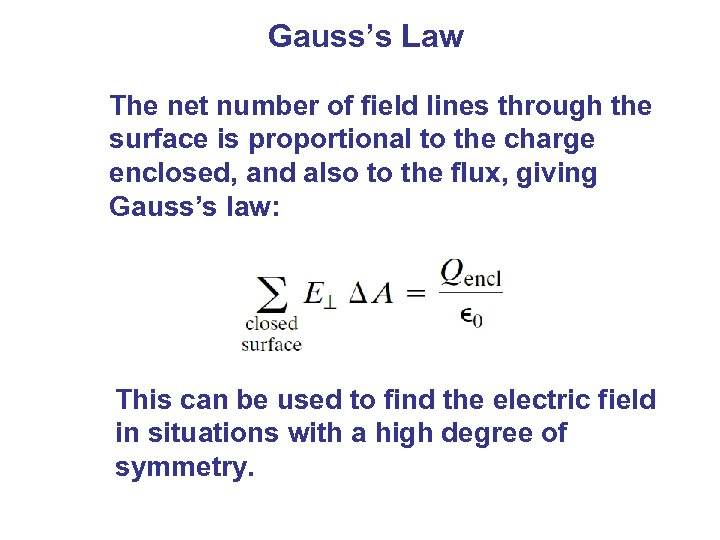Gauss’s Law The net number of field lines through the surface is proportional to the charge enclosed, and also to the flux, giving Gauss’s law: This can be used to find the electric field in situations with a high degree of symmetry.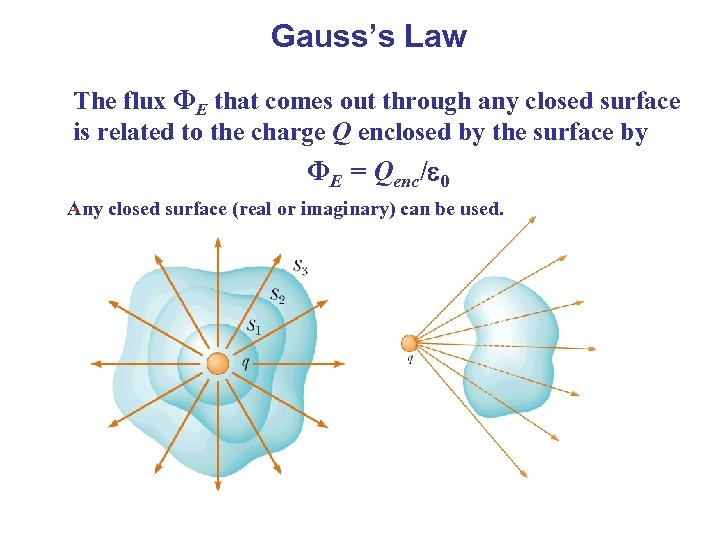Gauss’s Law The flux ΦE that comes out through any closed surface is related to the charge Q enclosed by the surface by ΦE = Qenc/ 0. Any closed surface (real or imaginary) can be used.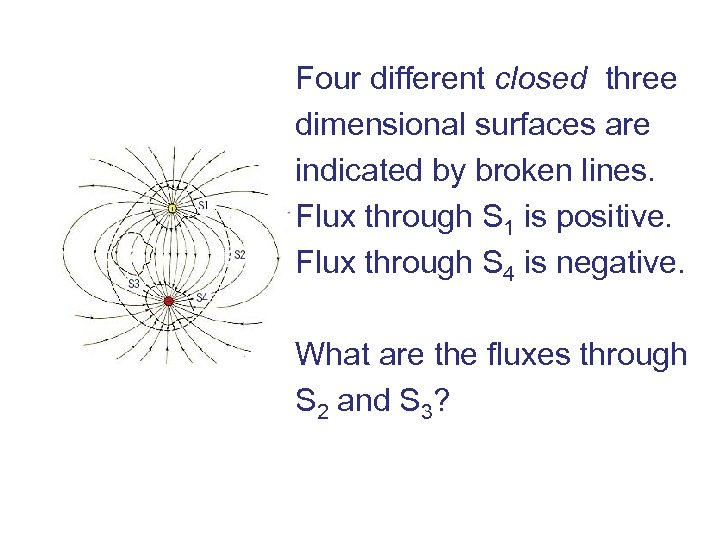Four different closed three dimensional surfaces are indicated by broken lines. Flux through S 1 is positive. Flux through S 4 is negative. What are the fluxes through S 2 and S 3?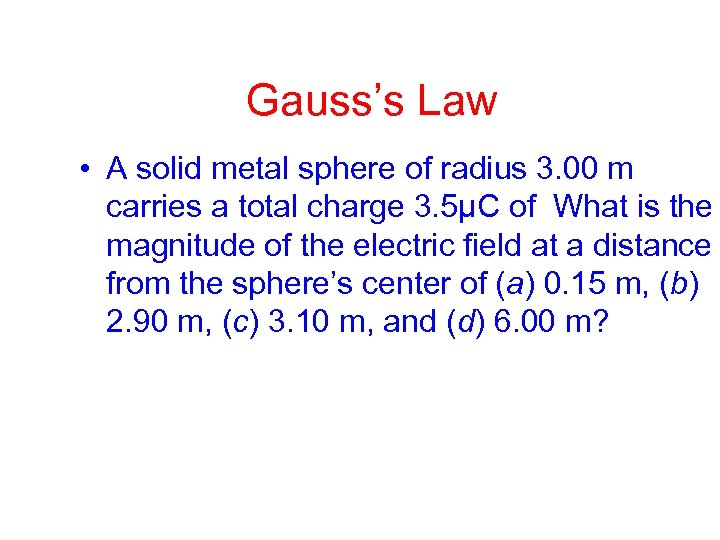Gauss’s Law • A solid metal sphere of radius 3. 00 m carries a total charge 3. 5μC of What is the magnitude of the electric field at a distance from the sphere’s center of (a) 0. 15 m, (b) 2. 90 m, (c) 3. 10 m, and (d) 6. 00 m?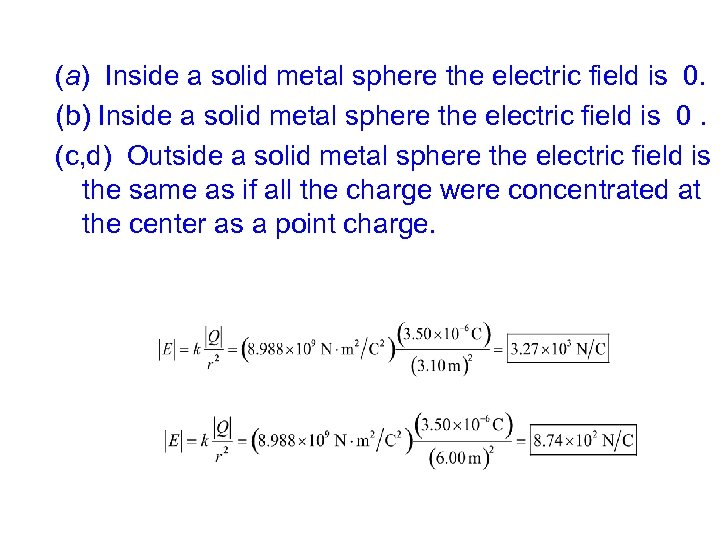(a) Inside a solid metal sphere the electric field is 0. (b) Inside a solid metal sphere the electric field is 0. (c, d) Outside a solid metal sphere the electric field is the same as if all the charge were concentrated at the center as a point charge.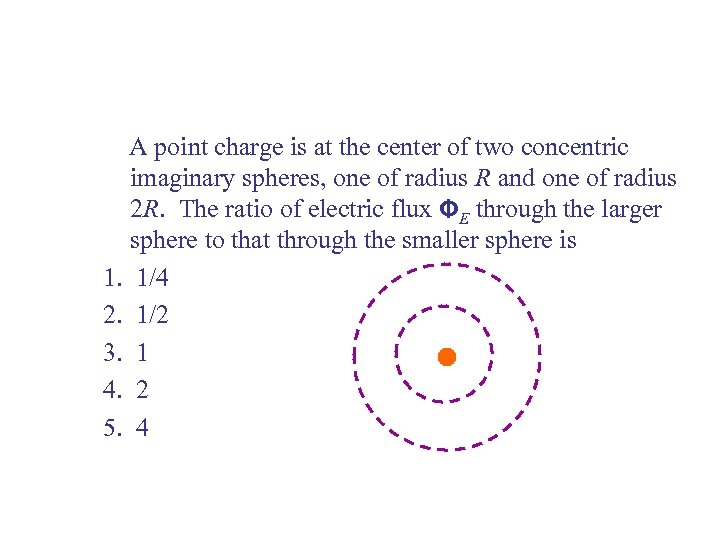A point charge is at the center of two concentric imaginary spheres, one of radius R and one of radius 2 R. The ratio of electric flux ΦE through the larger sphere to that through the smaller sphere is 1. 1/4 2. 1/2 3. 1 4. 2 5. 4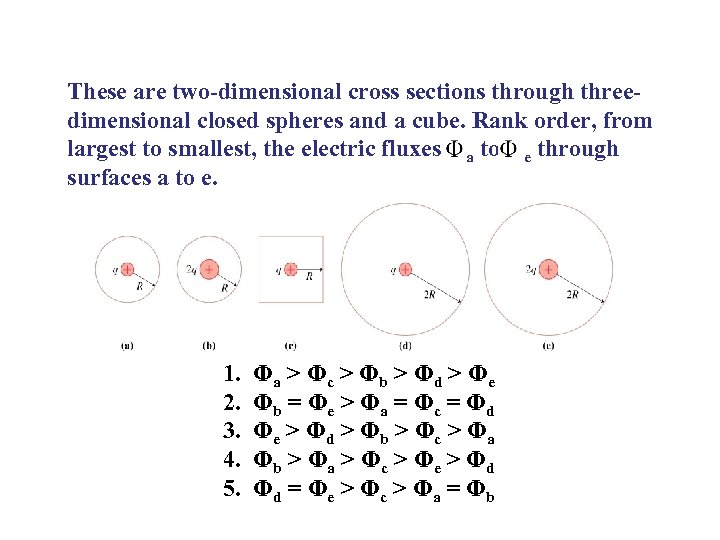These are two-dimensional cross sections through threedimensional closed spheres and a cube. Rank order, from largest to smallest, the electric fluxes a to e through surfaces a to e. 1. 2. 3. 4. 5. Φa > Φ c > Φ b > Φ d > Φ e Φb = Φ e > Φ a = Φ c = Φ d Φe > Φ d > Φ b > Φ c > Φ a Φb > Φ a > Φ c > Φ e > Φ d Φd = Φ e > Φ c > Φ a = Φ b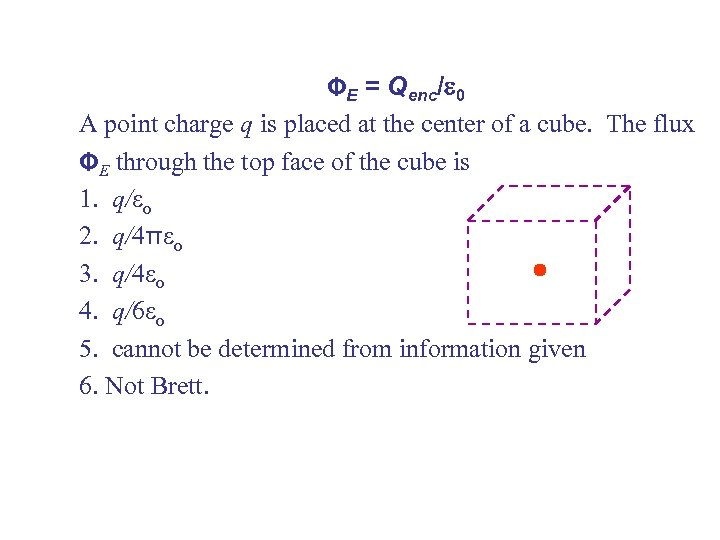ΦE = Qenc/ 0 A point charge q is placed at the center of a cube. The flux ΦE through the top face of the cube is 1. q/ o 2. q/4π o 3. q/4 o 4. q/6 o 5. cannot be determined from information given 6. Not Brett.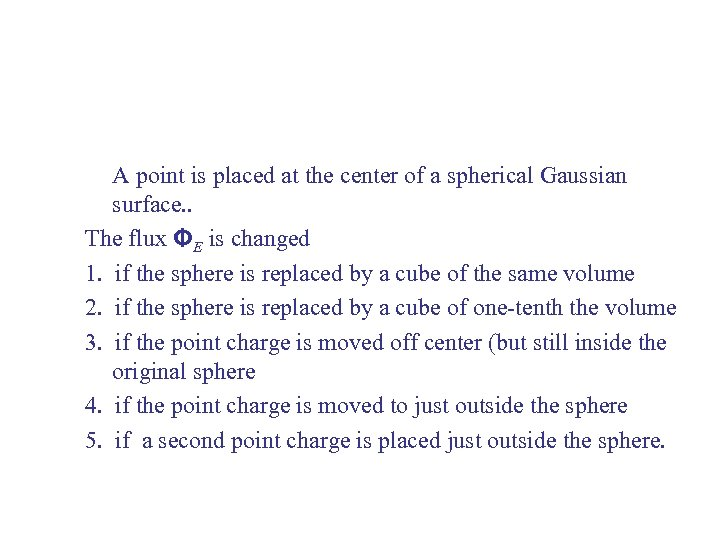A point is placed at the center of a spherical Gaussian surface. . The flux ΦE is changed 1. if the sphere is replaced by a cube of the same volume 2. if the sphere is replaced by a cube of one-tenth the volume 3. if the point charge is moved off center (but still inside the original sphere 4. if the point charge is moved to just outside the sphere 5. if a second point charge is placed just outside the sphere.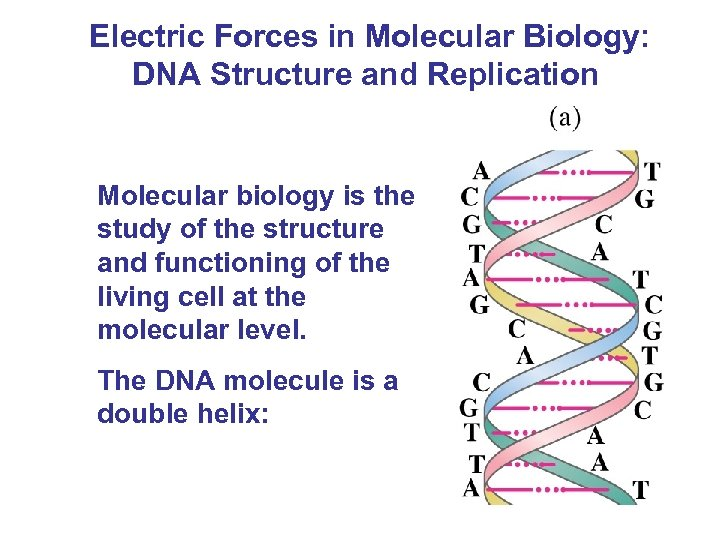Electric Forces in Molecular Biology: DNA Structure and Replication Molecular biology is the study of the structure and functioning of the living cell at the molecular level. The DNA molecule is a double helix: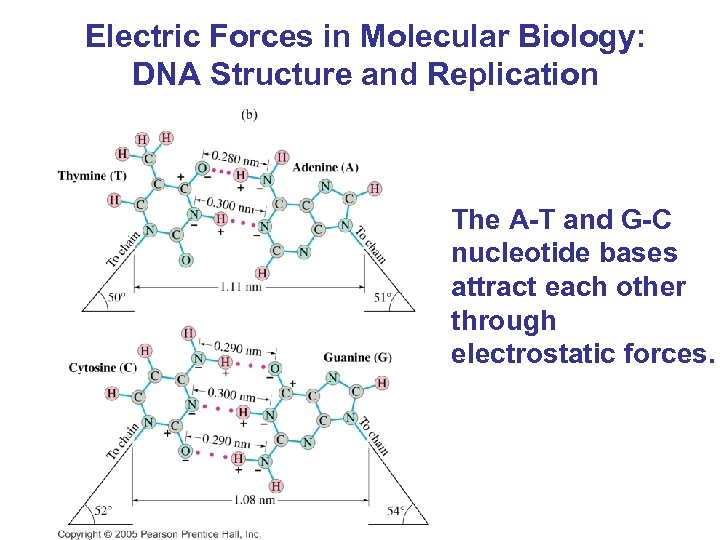Electric Forces in Molecular Biology: DNA Structure and Replication The A-T and G-C nucleotide bases attract each other through electrostatic forces.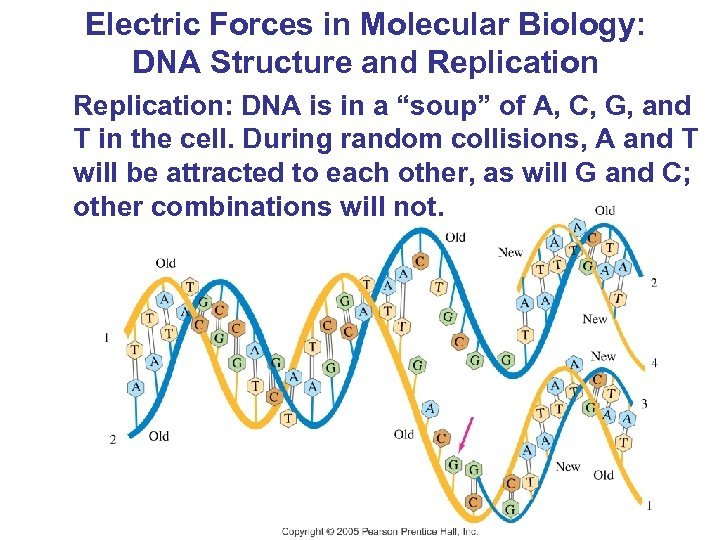Electric Forces in Molecular Biology: DNA Structure and Replication: DNA is in a “soup” of A, C, G, and T in the cell. During random collisions, A and T will be attracted to each other, as will G and C; other combinations will not.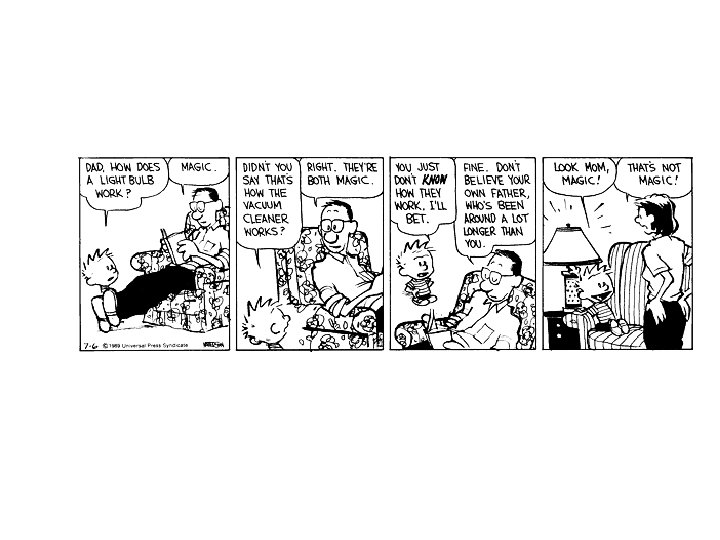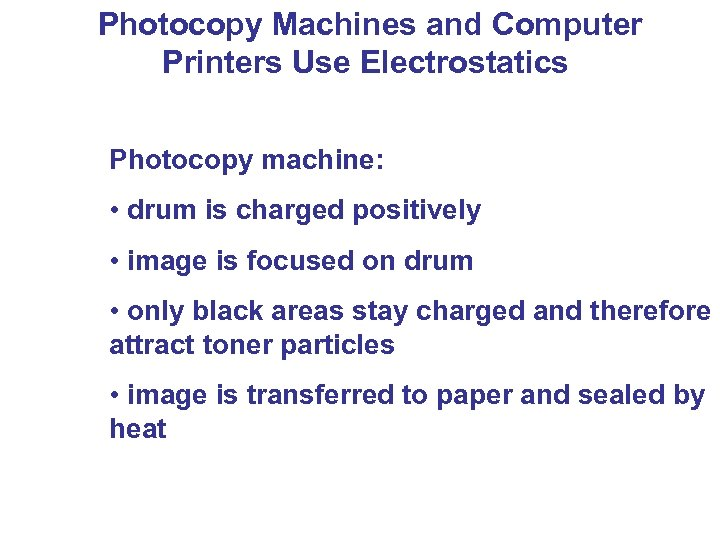Photocopy Machines and Computer Printers Use Electrostatics Photocopy machine: • drum is charged positively • image is focused on drum • only black areas stay charged and therefore attract toner particles • image is transferred to paper and sealed by heat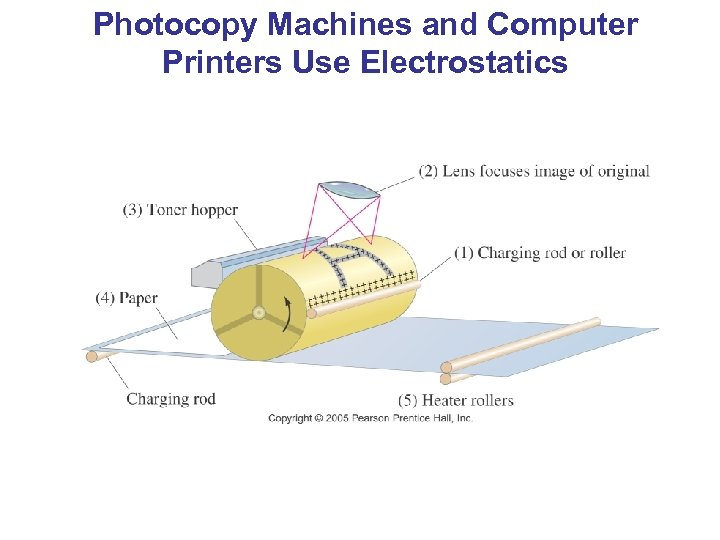Photocopy Machines and Computer Printers Use Electrostatics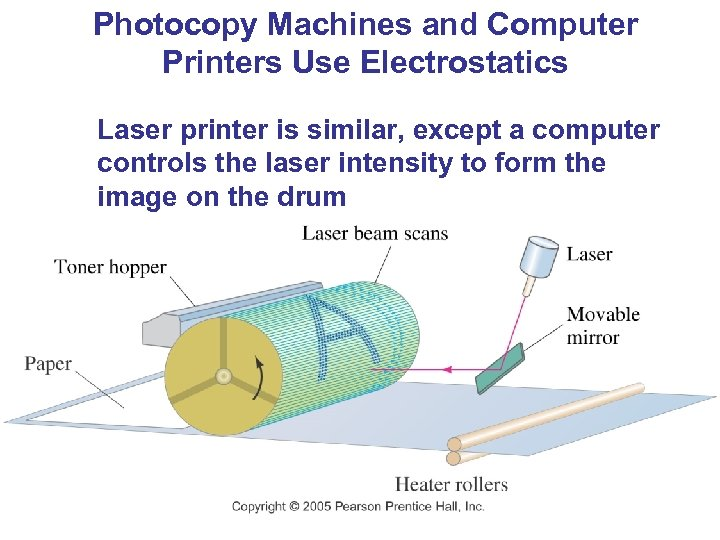Photocopy Machines and Computer Printers Use Electrostatics Laser printer is similar, except a computer controls the laser intensity to form the image on the drum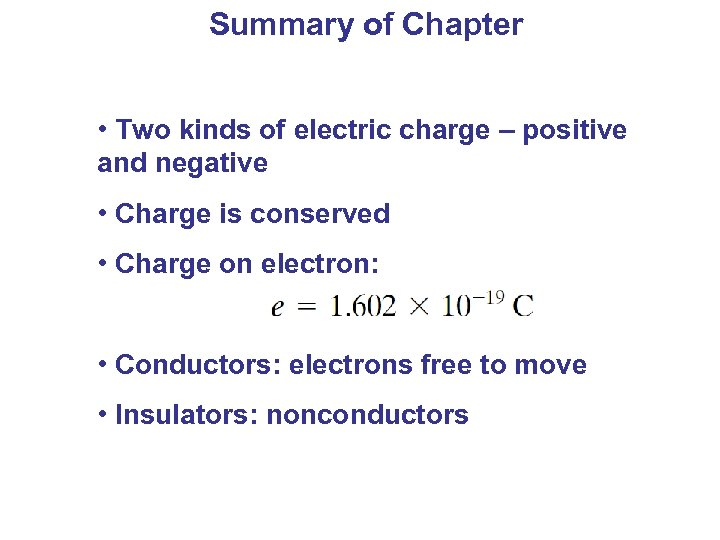Summary of Chapter • Two kinds of electric charge – positive and negative • Charge is conserved • Charge on electron: • Conductors: electrons free to move • Insulators: nonconductors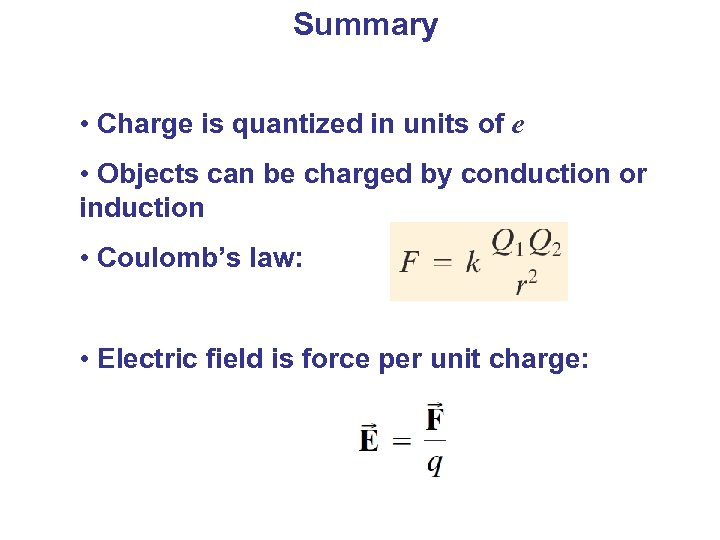Summary • Charge is quantized in units of e • Objects can be charged by conduction or induction • Coulomb’s law: • Electric field is force per unit charge: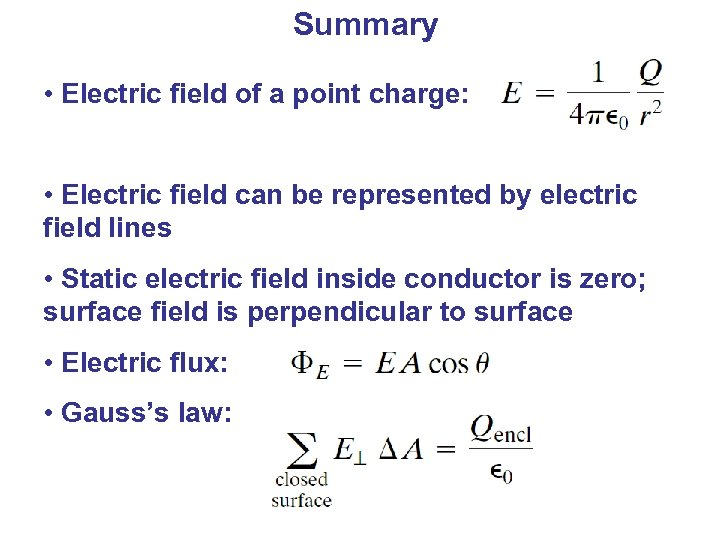Summary • Electric field of a point charge: • Electric field can be represented by electric field lines • Static electric field inside conductor is zero; surface field is perpendicular to surface • Electric flux: • Gauss’s law: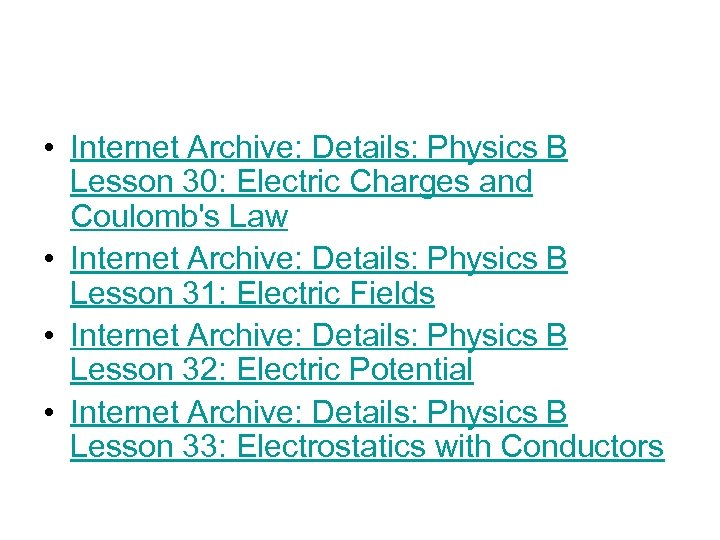• Internet Archive: Details: Physics B Lesson 30: Electric Charges and Coulomb's Law • Internet Archive: Details: Physics B Lesson 31: Electric Fields • Internet Archive: Details: Physics B Lesson 32: Electric Potential • Internet Archive: Details: Physics B Lesson 33: Electrostatics with Conductors